# NCERT Exemplar Class 12 Physics Solutions for Chapter 3 - Current Electricity

NCERT Exemplar Solutions for Class 12 Physics Chapter 3 Current Electricity are provided here to help you gain complete knowledge on the topics covered in this chapter. NCERT Exemplar for Class 12 Physics Chapter 3 PDF contains solutions to the exemplar problems given in the NCERT book, along with extra questions from previous years’ question papers and sample papers, worksheets, MCQs and short and long answer questions, etc.

## Download the PDF of NCERT Exemplar Solutions for Class 12 Physics Chapter 3 Current Electricity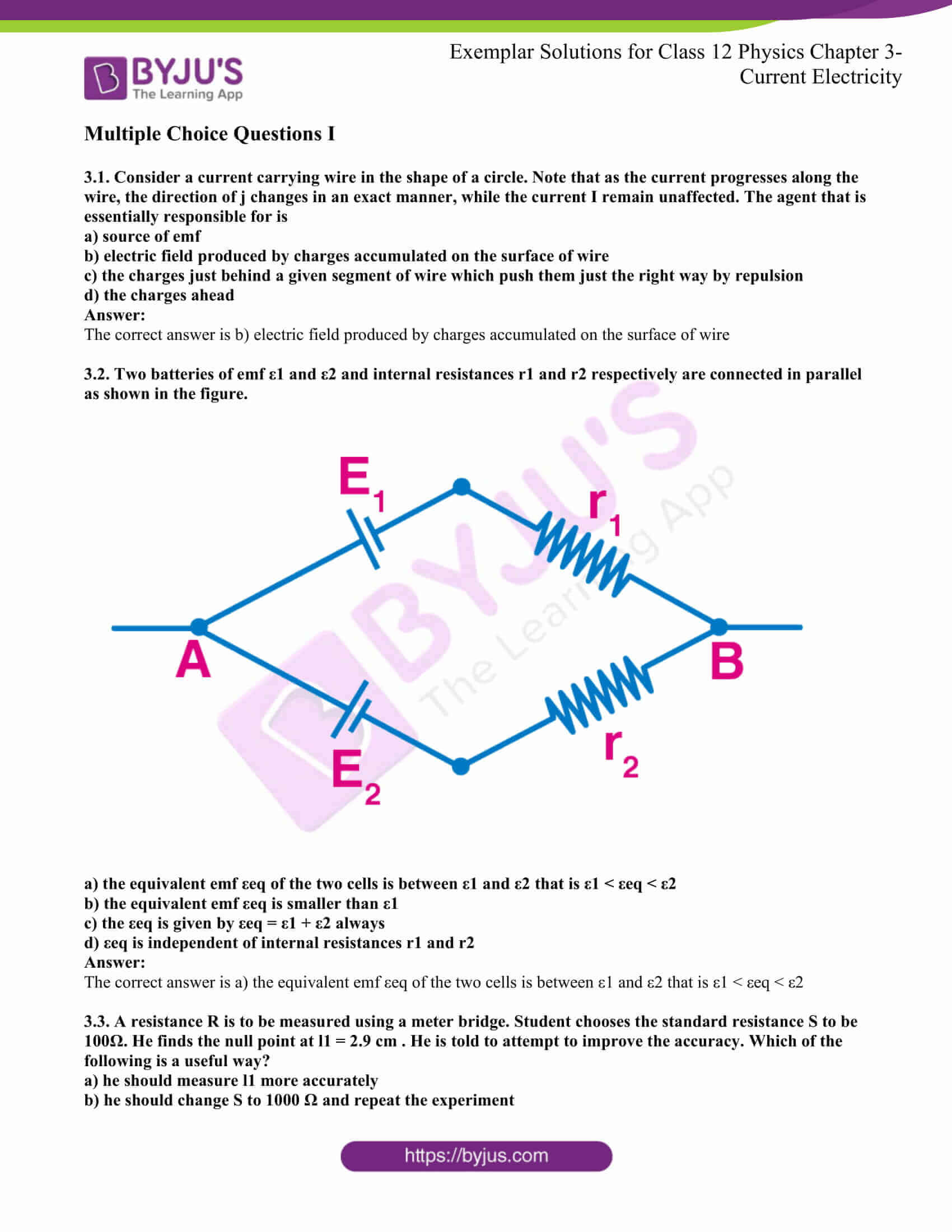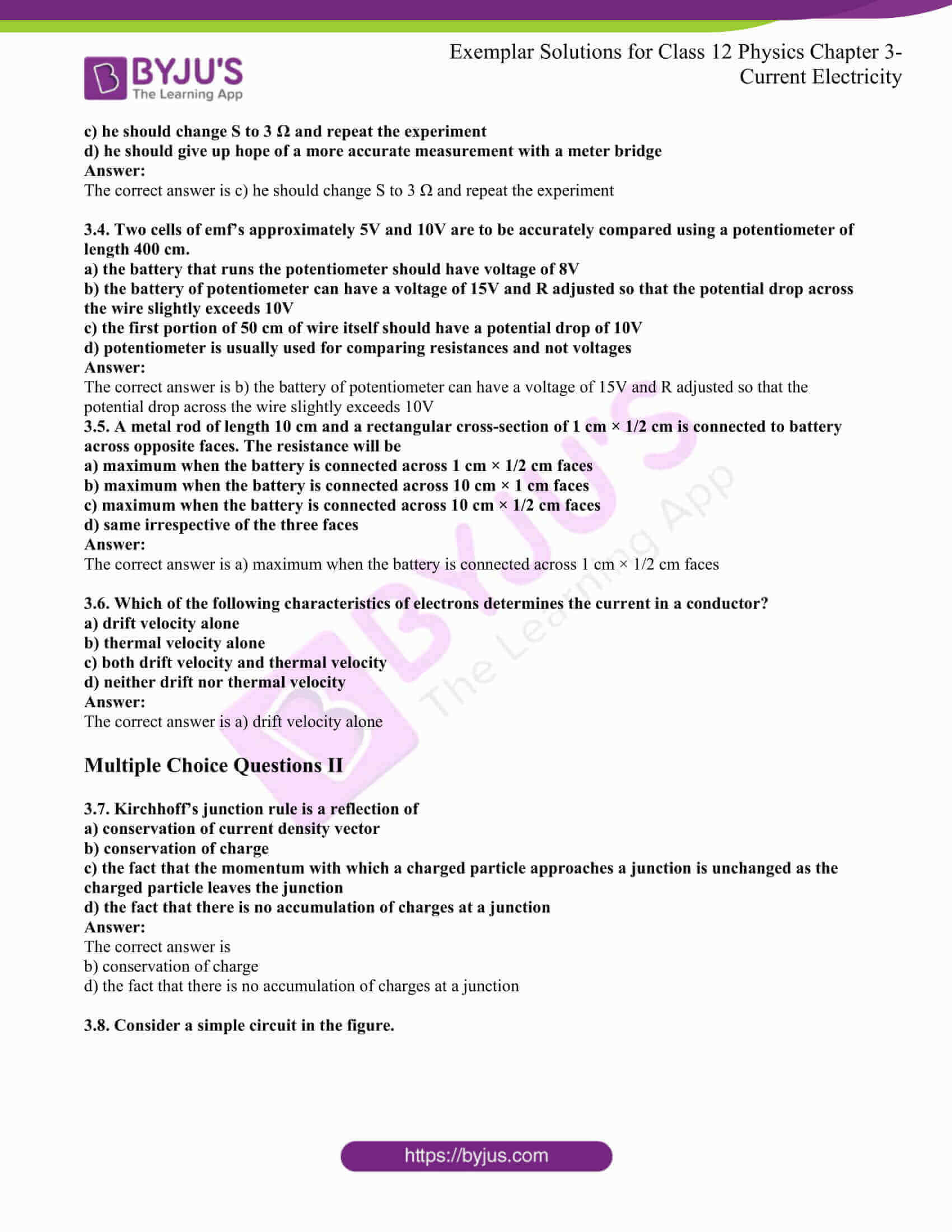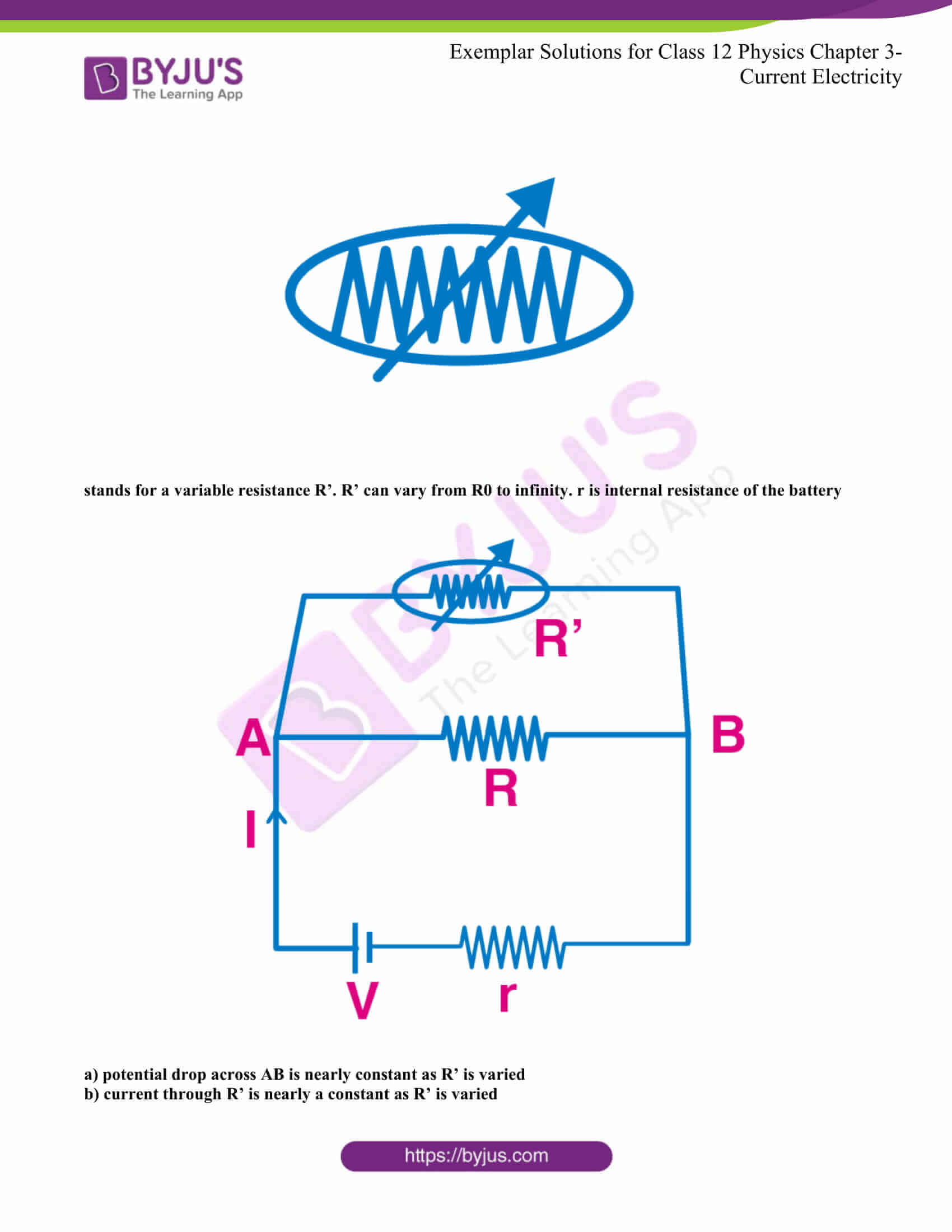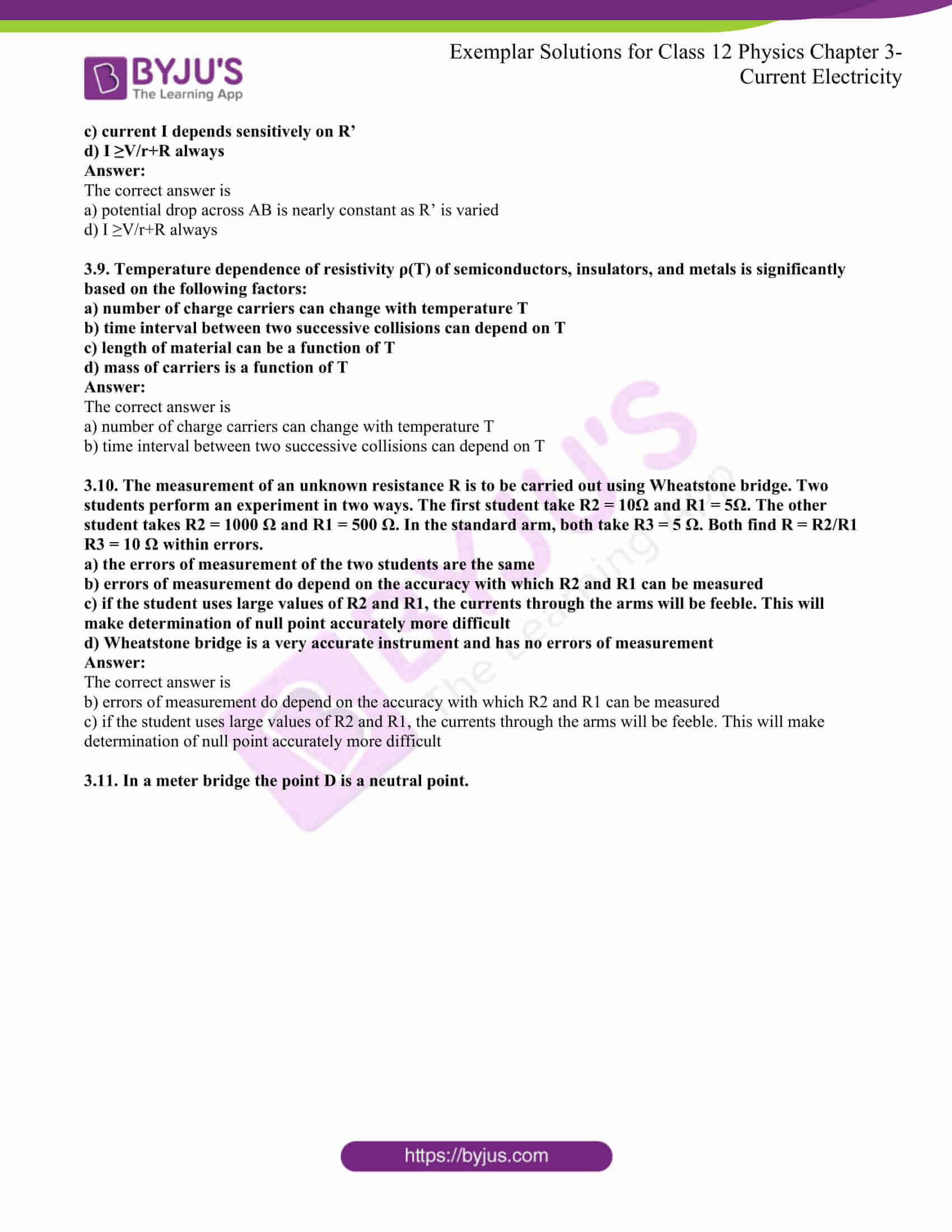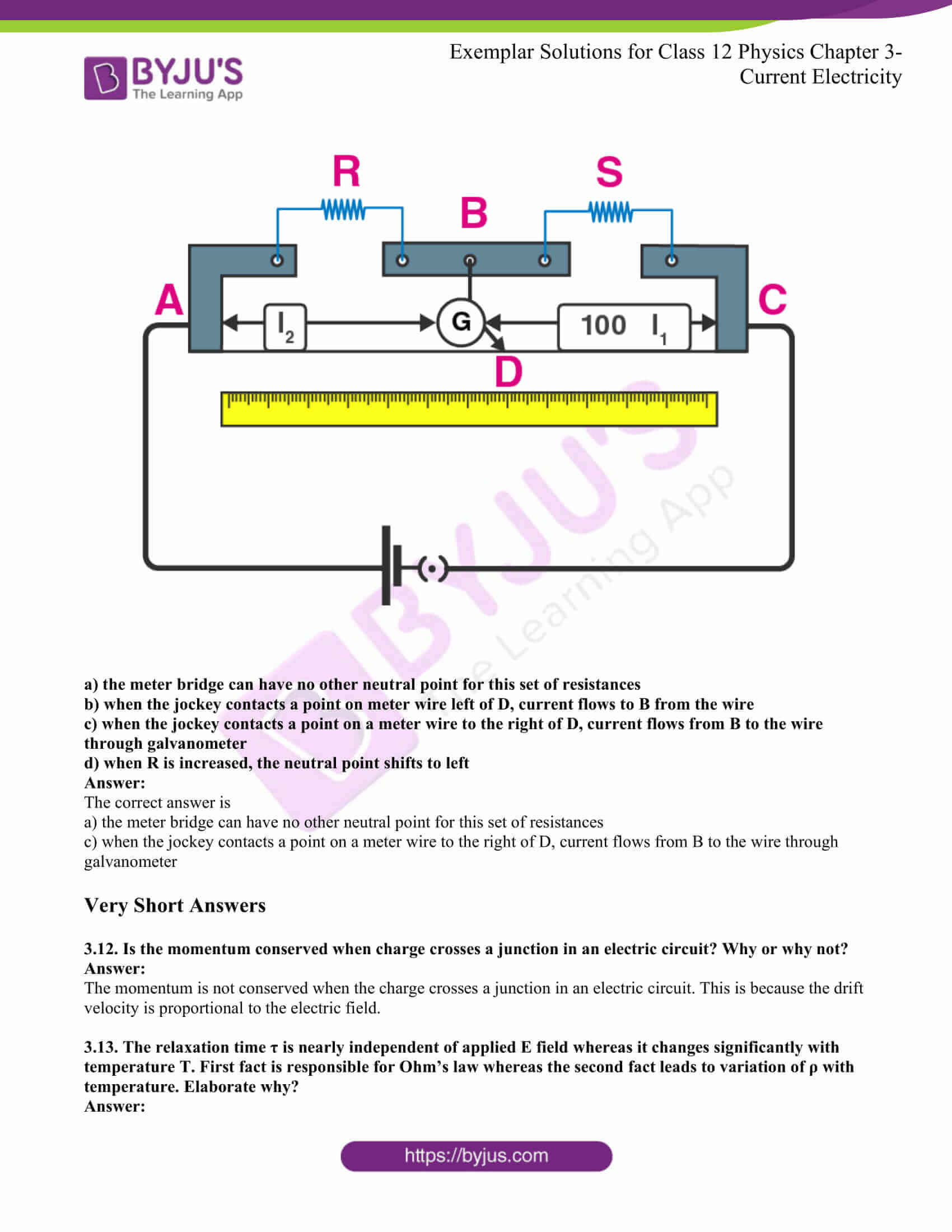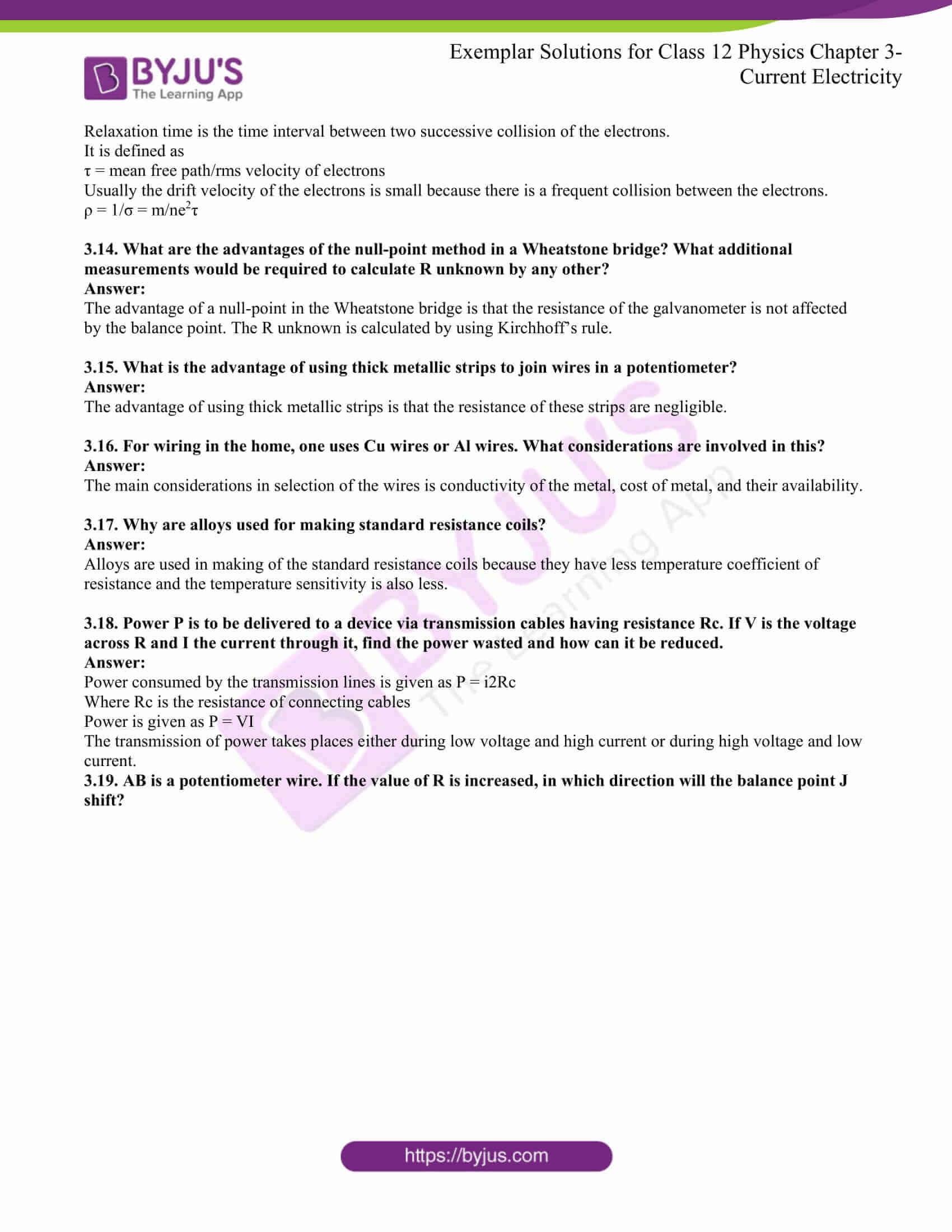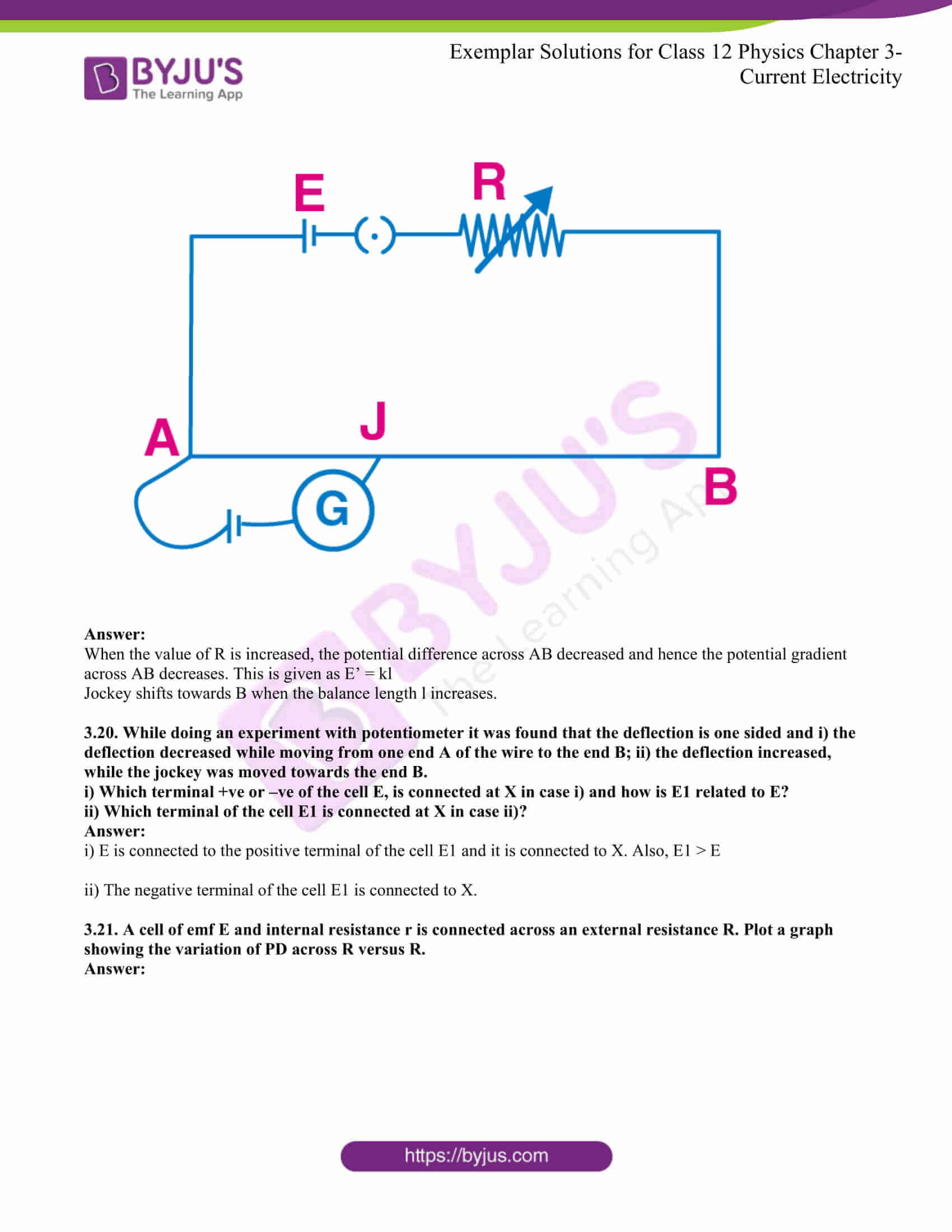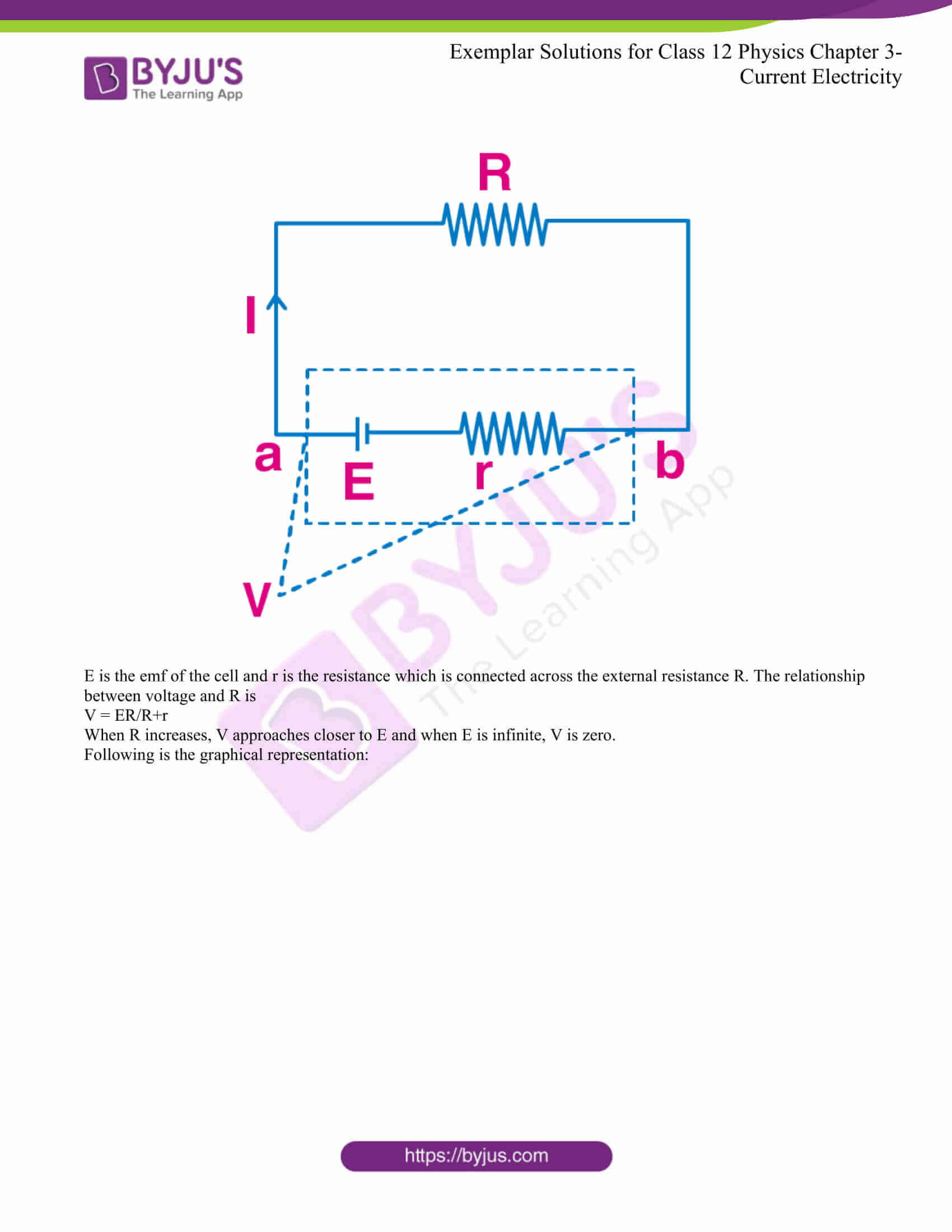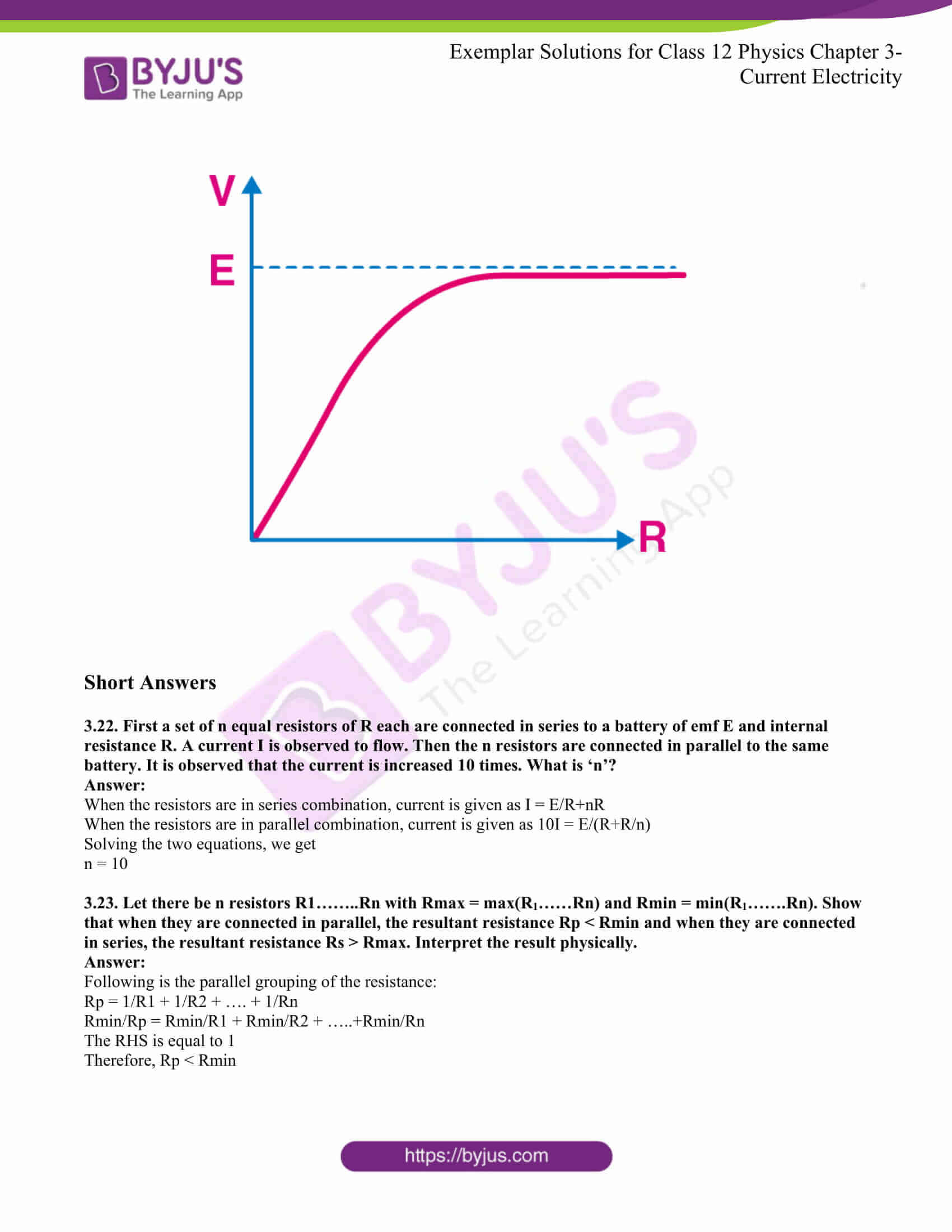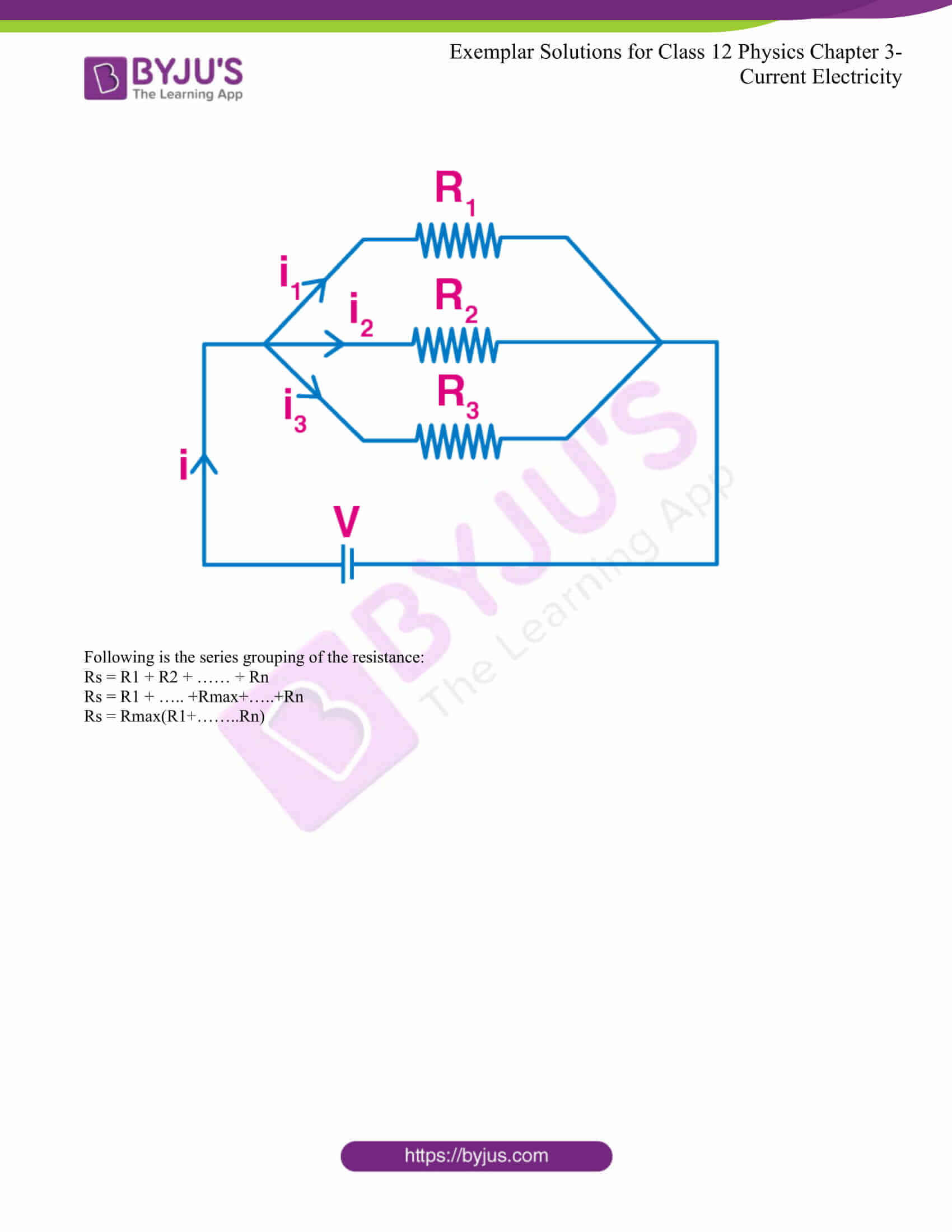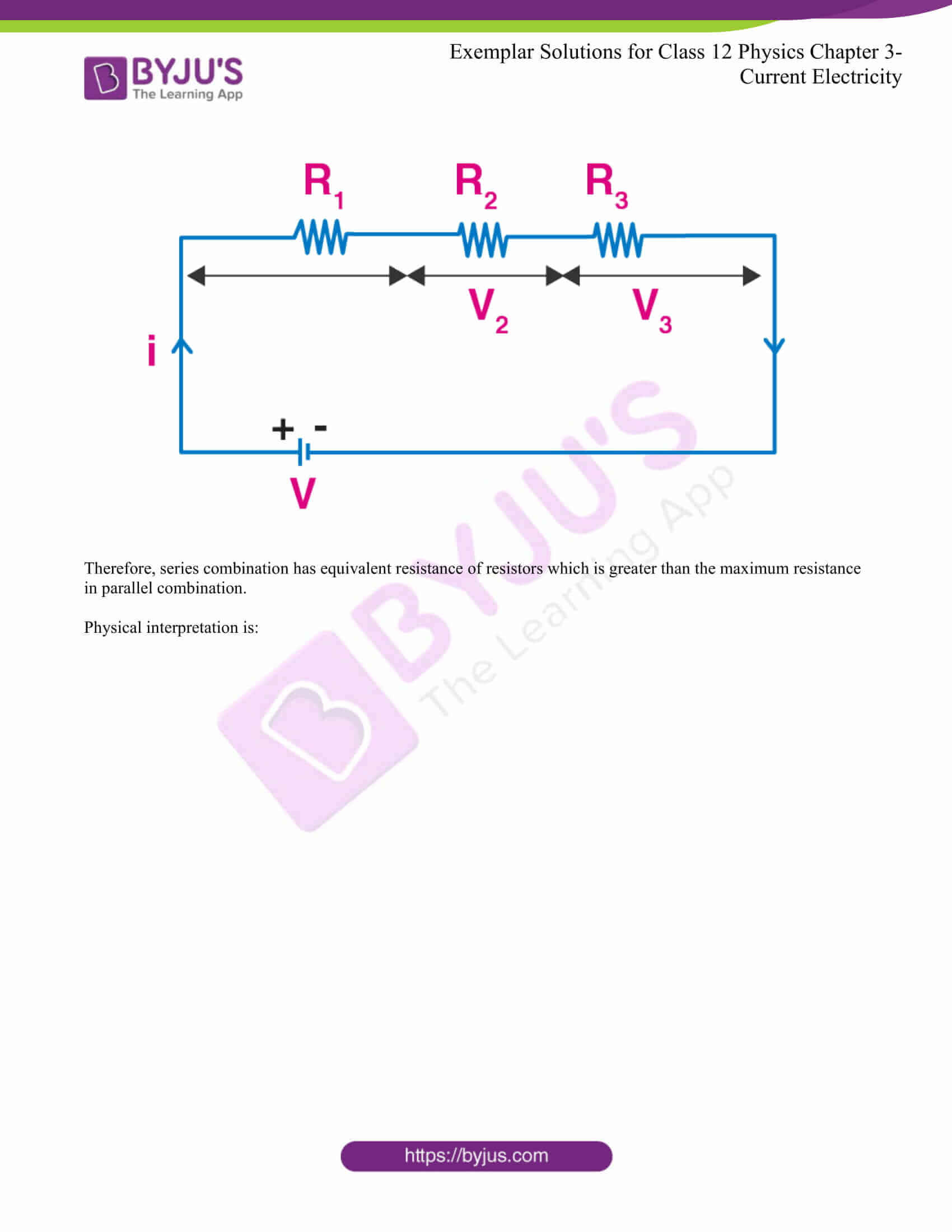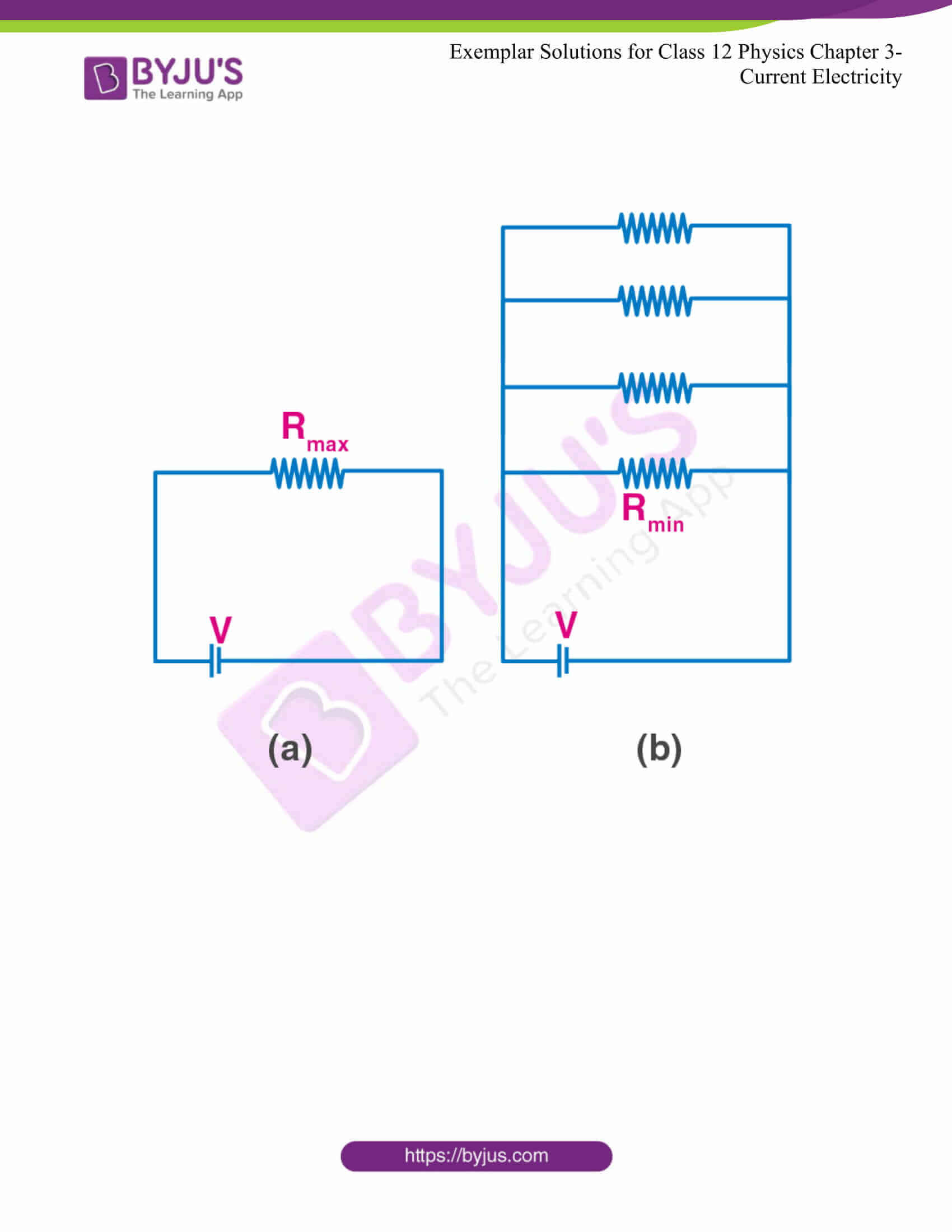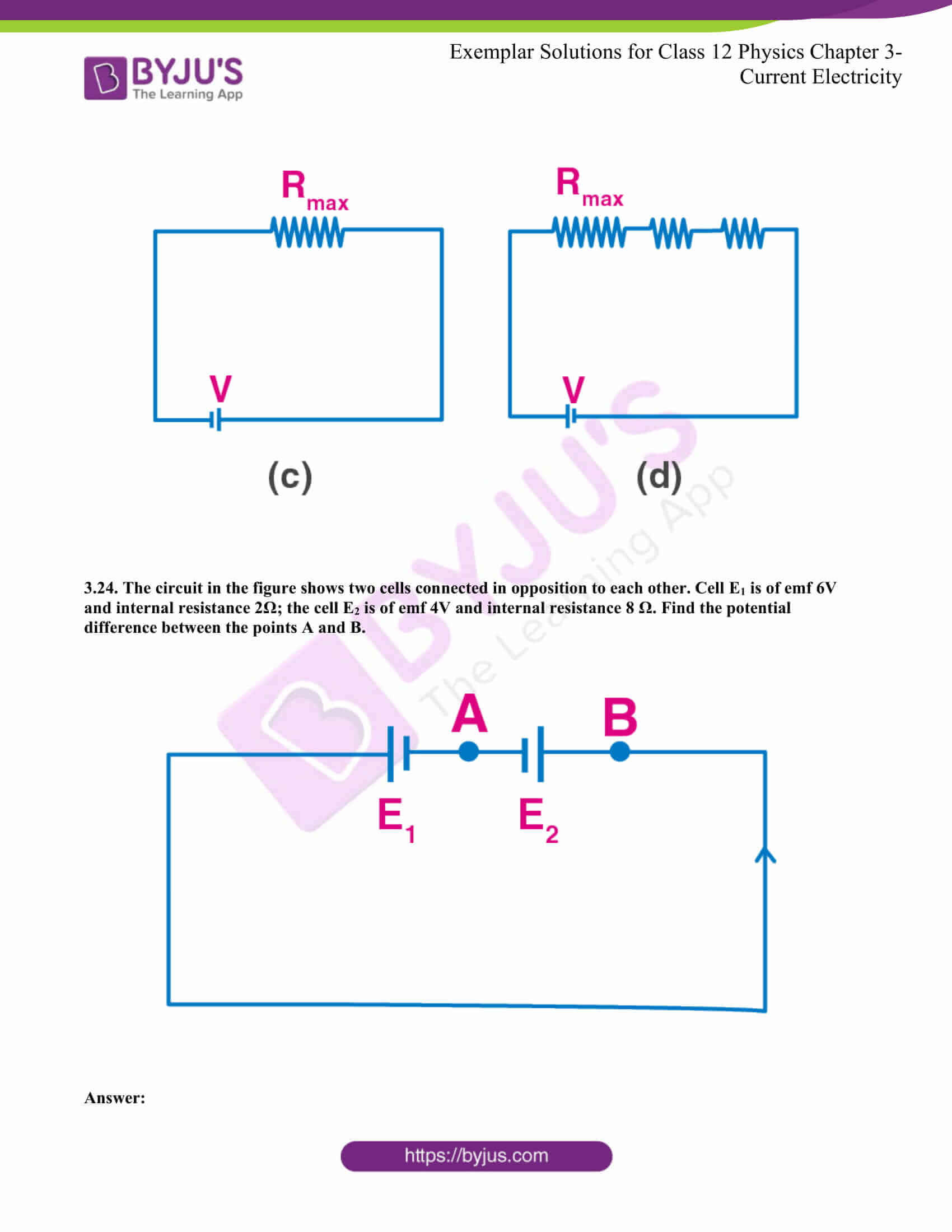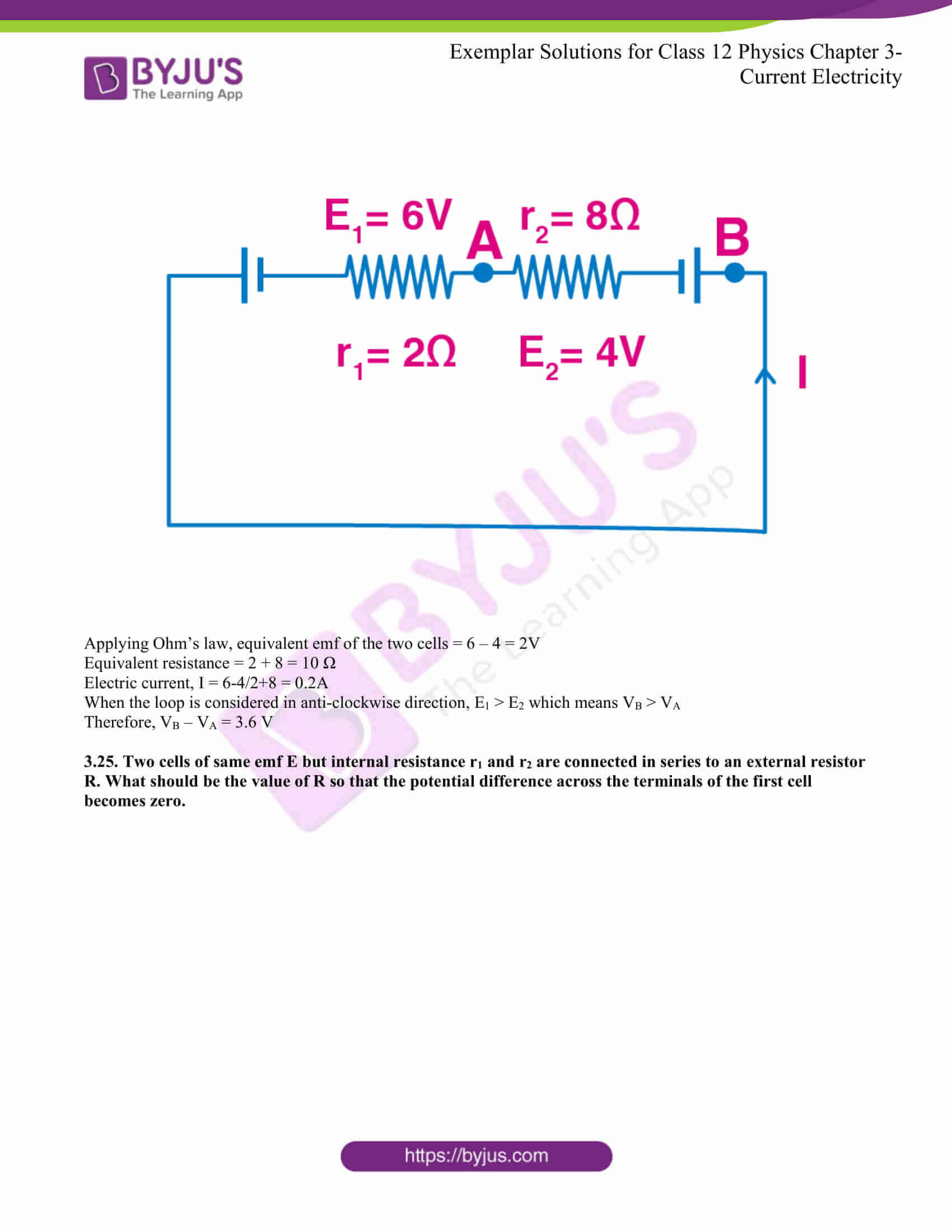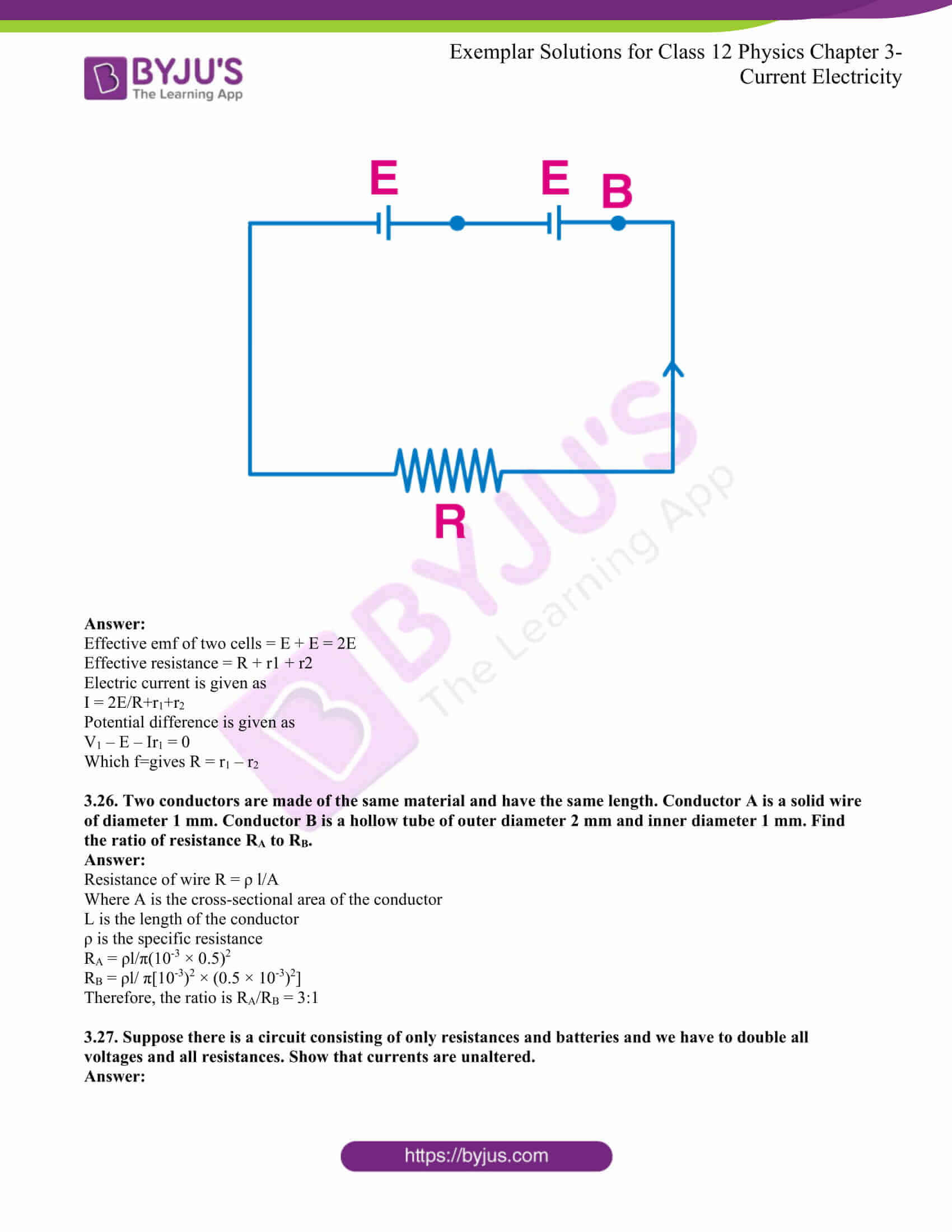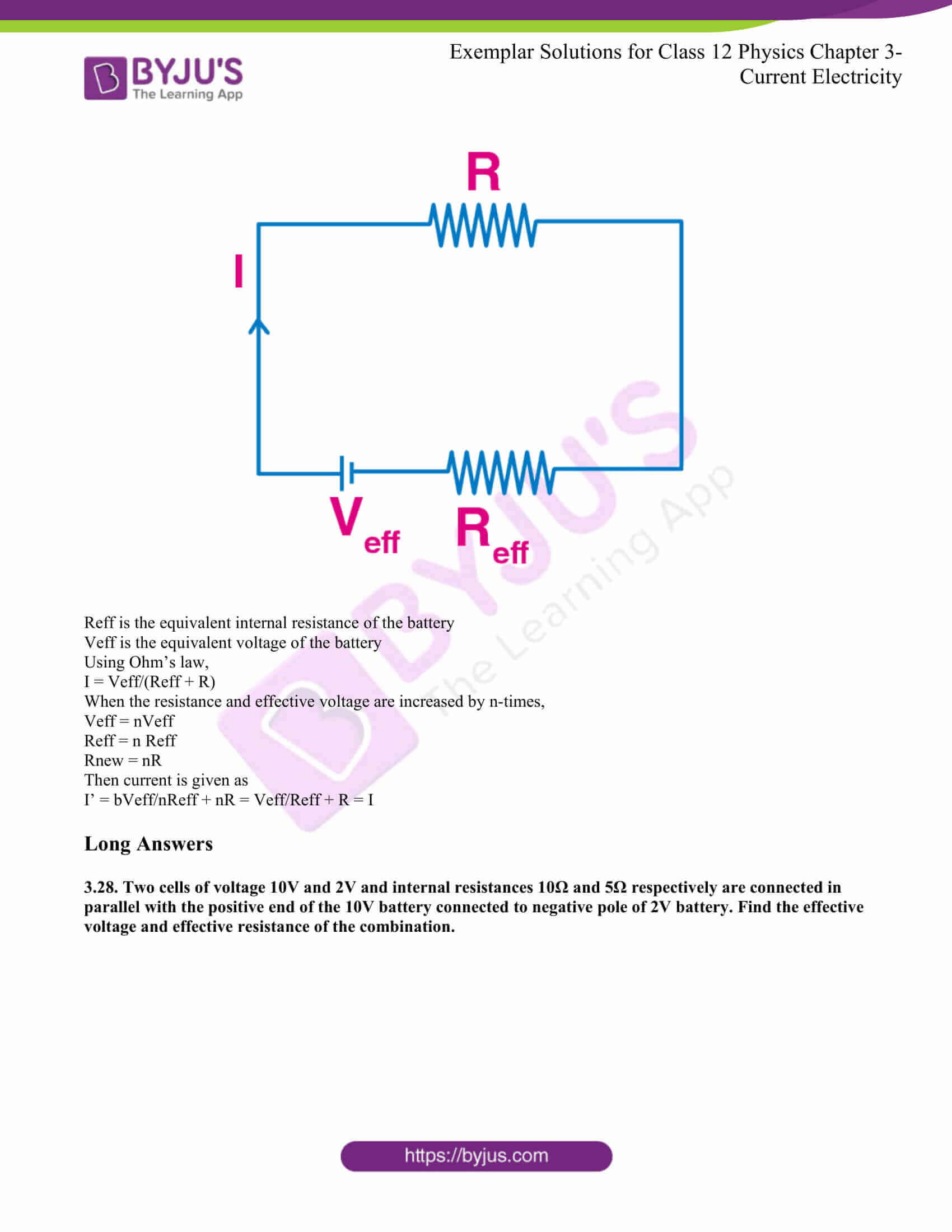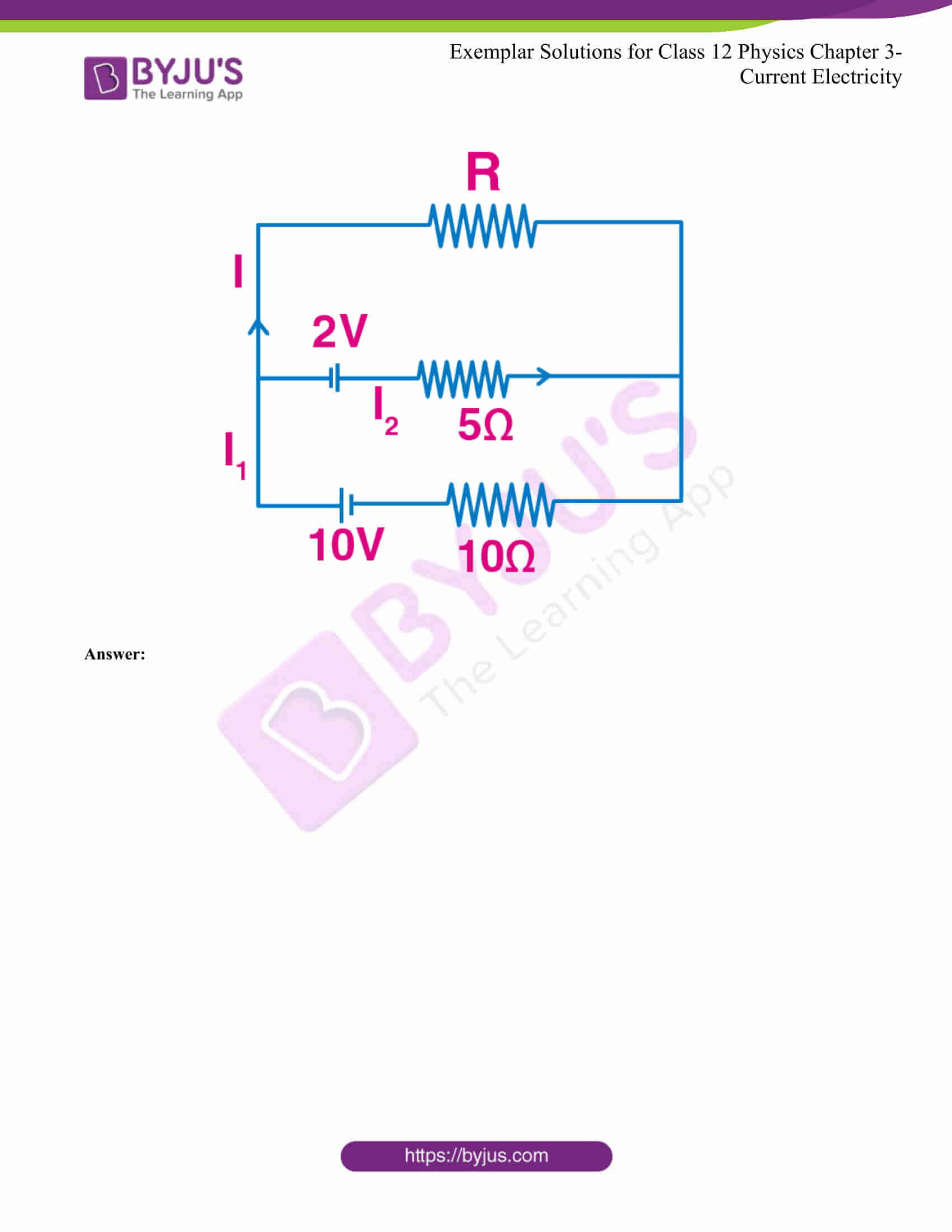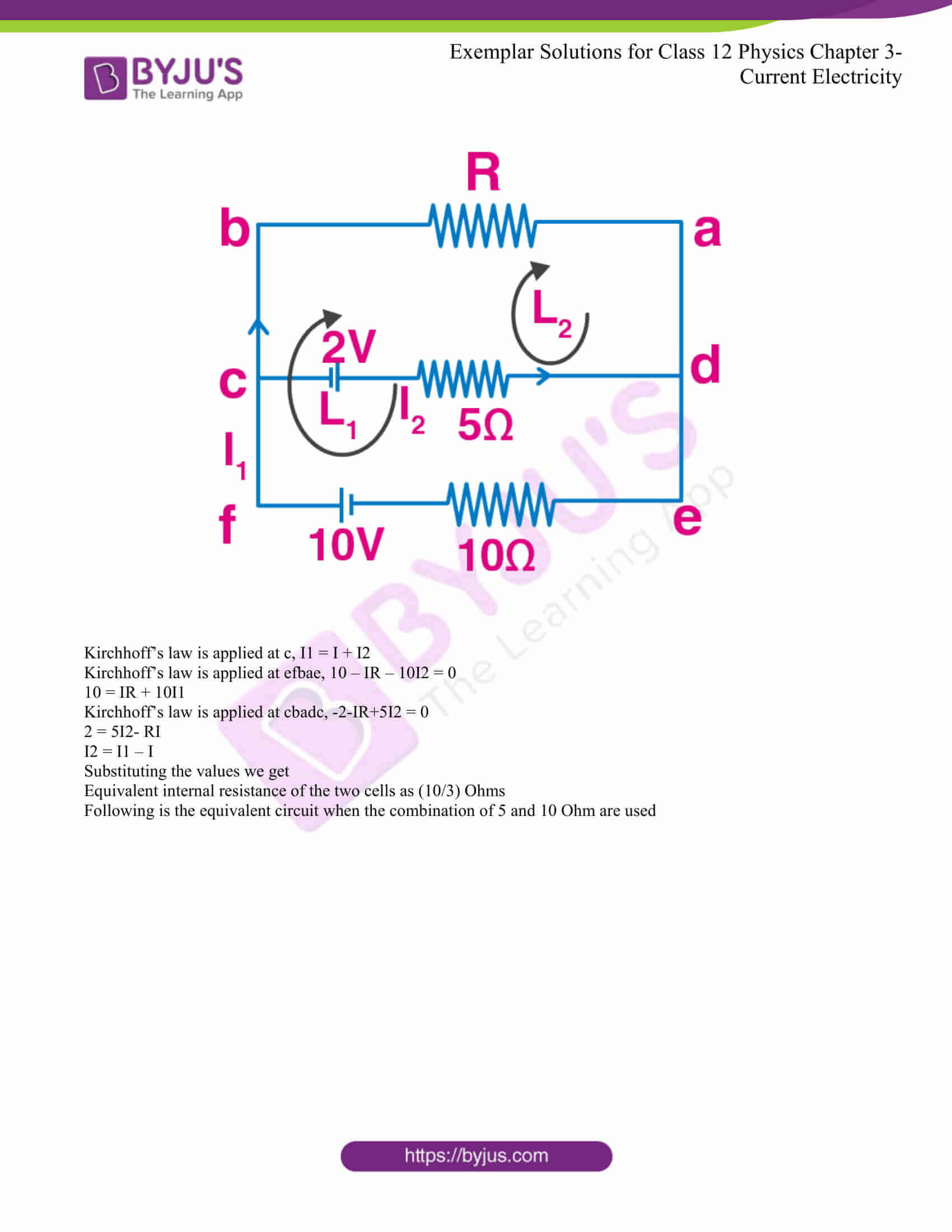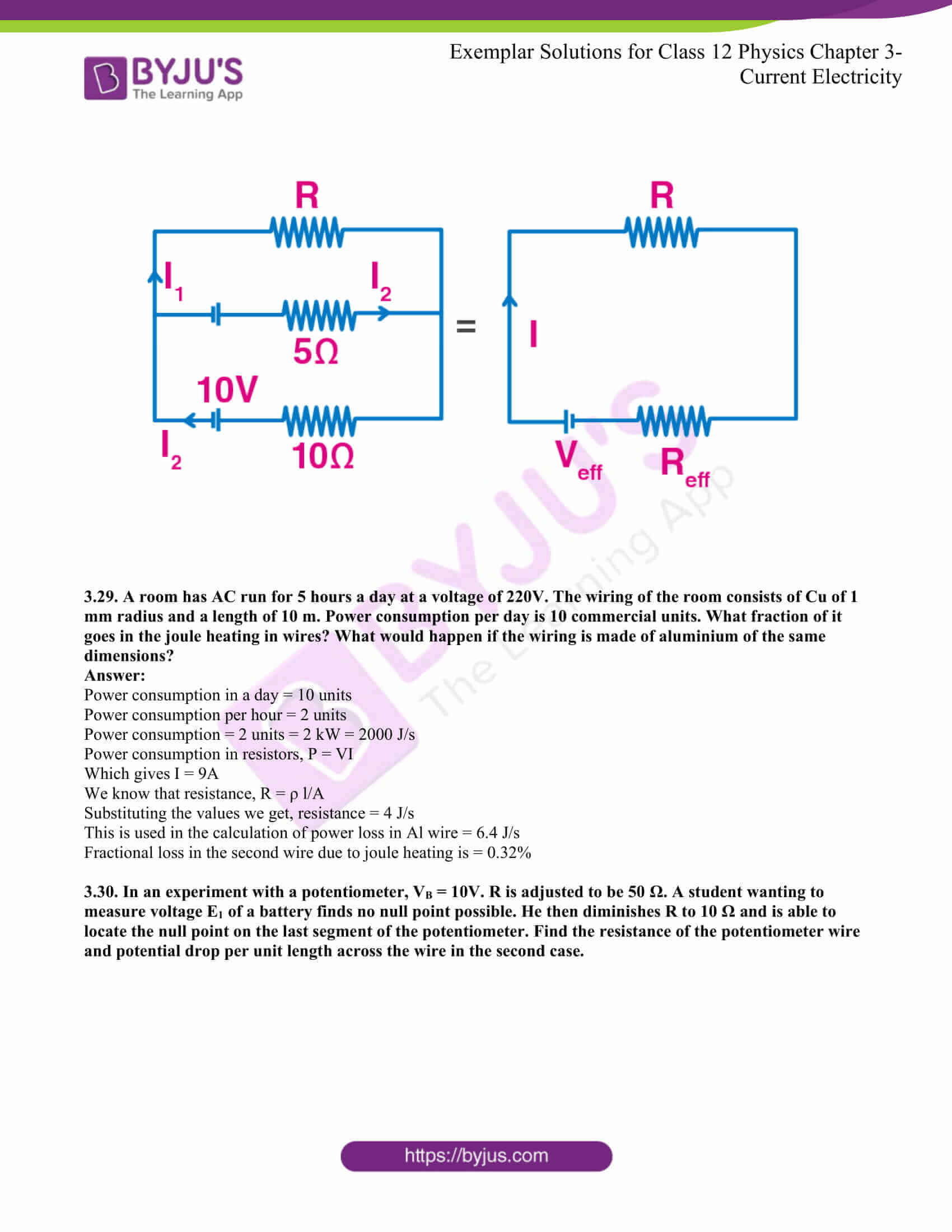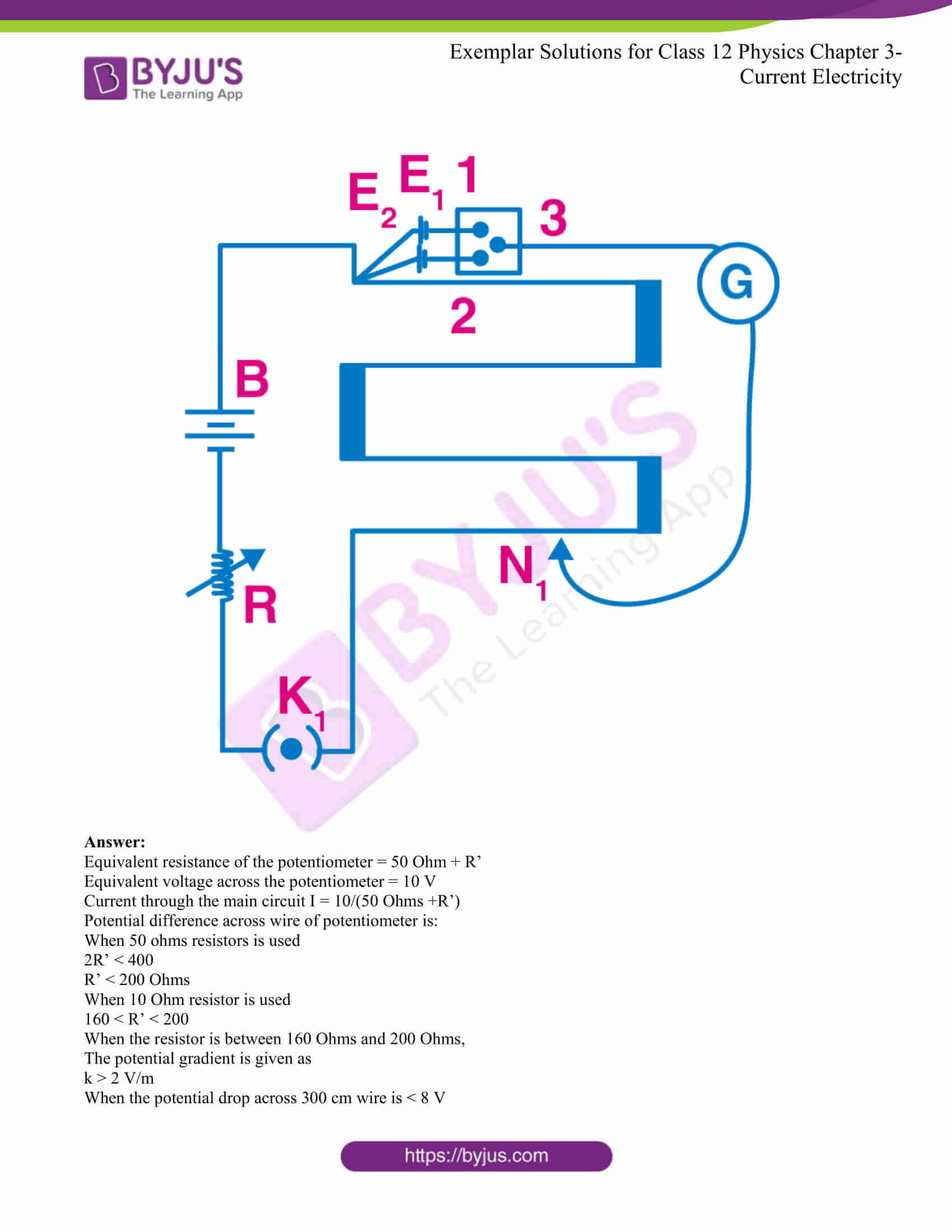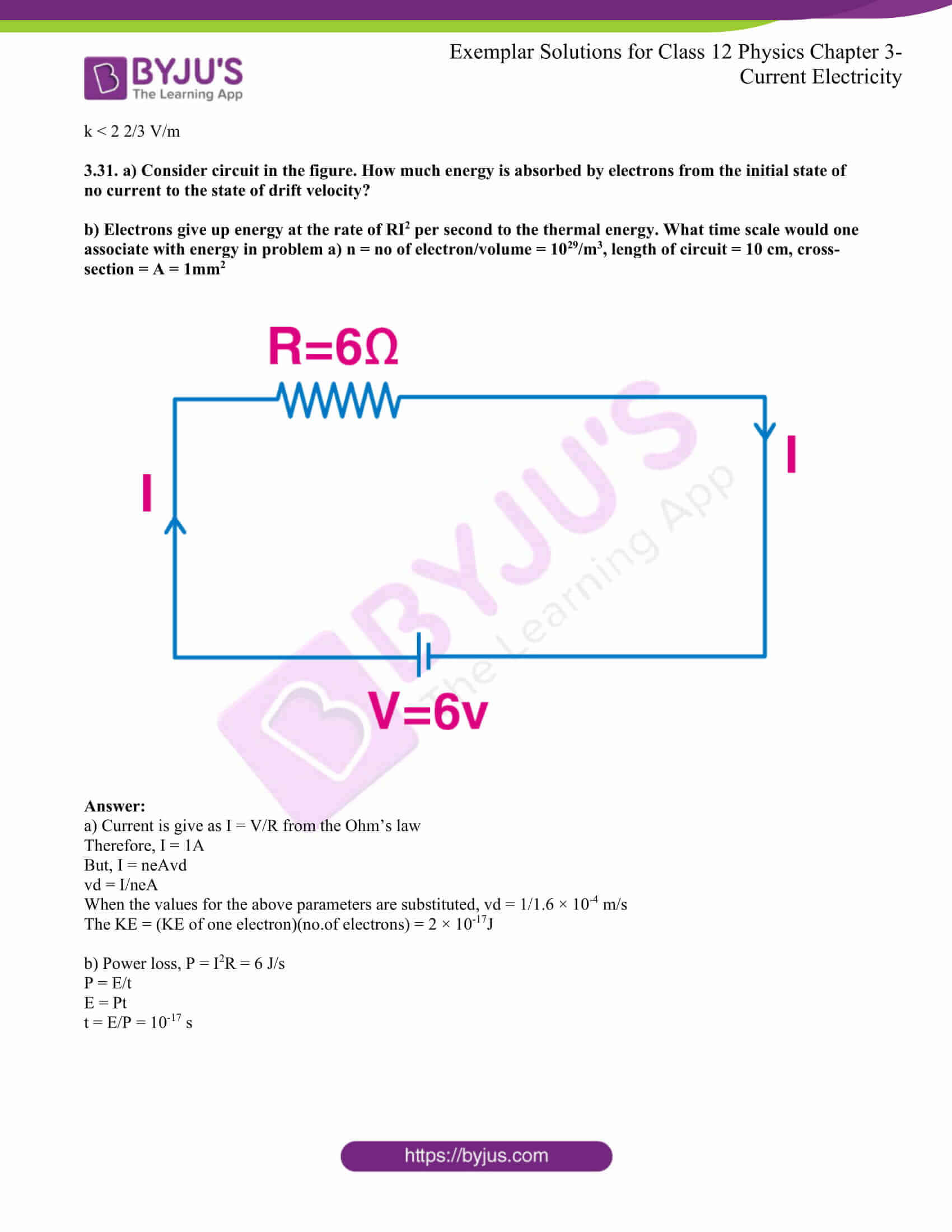### Multiple-choice Questions I

3.1. Consider a current-carrying wire in the shape of a circle. Note that as the current progresses along the wire, the direction of j changes in an exact manner, while the current I remain unaffected. The agent that is essentially responsible for this is

a) source of emf

b) electric field produced by charges accumulated on the surface of the wire

c) the charges just behind a given segment of wire which push them just the right way by repulsion

The correct answer is b) electric field produced by charges accumulated on the surface of the wire

3.2. Two batteries of emf ε1 and ε2 and internal resistances r1 and r2, respectively, are connected in parallel, as shown in the figure.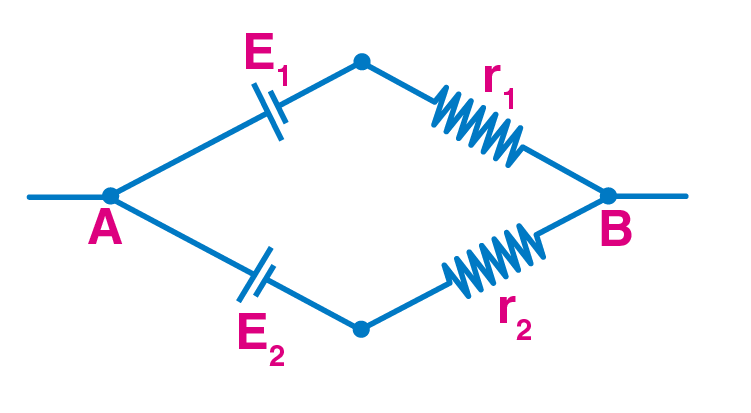a) the equivalent emf εeq of the two cells is between ε1 and ε2 that is ε1 < εeq < ε2

b) the equivalent emf εeq is smaller than ε1

c) the εeq is given by εeq = ε1 + ε2 always

d) εeq is independent of internal resistances r1 and r2

The correct answer is a) the equivalent emf εeq of the two cells is between ε1 and ε2 that is ε1 < εeq < ε2

3.3. A resistance R is to be measured using a meter bridge. The student chooses the standard resistance S to be 100Ω. He finds the null point at l1 = 2.9 cm. He is told to attempt to improve accuracy. Which of the following is a useful way?

a) he should measure l1 more accurately

b) he should change S to 1000 Ω and repeat the experiment

c) he should change S to 3 Ω and repeat the experiment

d) he should give up hope of a more accurate measurement with a meter bridge

The correct answer is c) he should change S to 3 Ω and repeat the experiment

3.4. Two cells of emf’s, approximately 5V and 10V, are to be accurately compared using a potentiometer of length 400 cm.

a) the battery that runs the potentiometer should have voltage of 8V

b) the battery of potentiometer can have a voltage of 15V and R adjusted so that the potential drop across the wire slightly exceeds 10V

c) the first portion of 50 cm of wire itself should have a potential drop of 10V

d) potentiometer is usually used for comparing resistances and not voltages

The correct answer is b) the battery of potentiometer can have a voltage of 15V and R adjusted so that the potential drop across the wire slightly exceeds 10V

3.5. A metal rod of length 10 cm and a rectangular cross-section of 1 cm × 1/2 cm is connected to battery across opposite faces. The resistance will be

a) maximum when the battery is connected across 1 cm × 1/2 cm faces

b) maximum when the battery is connected across 10 cm × 1 cm faces

c) maximum when the battery is connected across 10 cm × 1/2 cm faces

d) same irrespective of the three faces

The correct answer is a) maximum when the battery is connected across 1 cm × 1/2 cm faces

3.6. Which of the following characteristics of electrons determines the current in a conductor?

a) drift velocity alone

b) thermal velocity alone

c) both drift velocity and thermal velocity

d) neither drift nor thermal velocity

The correct answer is a) drift velocity alone

### Multiple Choice Questions II

3.7. Kirchhoff’s junction rule is a reflection of

a) conservation of current density vector

b) conservation of charge

c) the fact that the momentum with which a charged particle approaches a junction is unchanged as the charged particle leaves the junction

d) the fact that there is no accumulation of charges at a junction

b) conservation of charge

d) the fact that there is no accumulation of charges at a junction

3.8. Consider a simple circuit in the figure.stands for a variable resistance R’. R’ can vary from R0 to infinity. r is internal resistance of the battery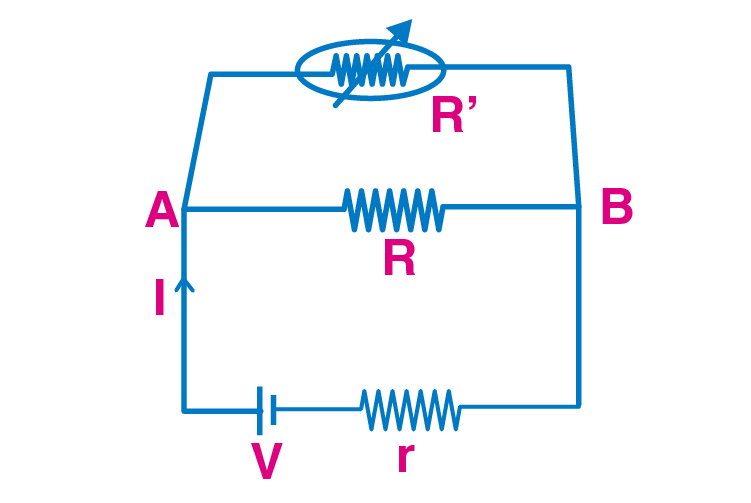a) potential drop across AB is nearly constant as R’ is varied

b) current through R’ is nearly a constant as R’ is varied

c) current I depends sensitively on R’

d) I ≥V/r+R always

a) potential drop across AB is nearly constant as R’ is varied

d) I ≥V/r+R always

3.9. Temperature dependence of resistivity ρ(T) of semiconductors, insulators, and metals is significantly based on the following factors:

a) number of charge carriers can change with temperature T

b) time interval between two successive collisions can depend on T

c) length of material can be a function of T

d) mass of carriers is a function of T

a) number of charge carriers can change with temperature T

b) time interval between two successive collisions can depend on T

3.10. The measurement of an unknown resistance R is to be carried out using a Wheatstone bridge. Two students perform an experiment in two ways. The first student takes R2 = 10Ω and R1 = 5Ω. The other student takes R2 = 1000 Ω and R1 = 500 Ω. In the standard arm, both take R3 = 5 Ω. Both find R = R2/R1 R3 = 10 Ω within errors.

a) the errors of measurement of the two students are the same

b) errors of measurement do depend on the accuracy with which R2 and R1 can be measured

c) if the student uses large values of R2 and R1, the currents through the arms will be feeble. This will make determination of null point accurately more difficult

d) Wheatstone bridge is a very accurate instrument and has no errors of measurement

b) errors of measurement do depend on the accuracy with which R2 and R1 can be measured

c) if the student uses large values of R2 and R1, the currents through the arms will be feeble. This will make determination of null point accurately more difficult

3.11. In a meter bridge, point D is a neutral point.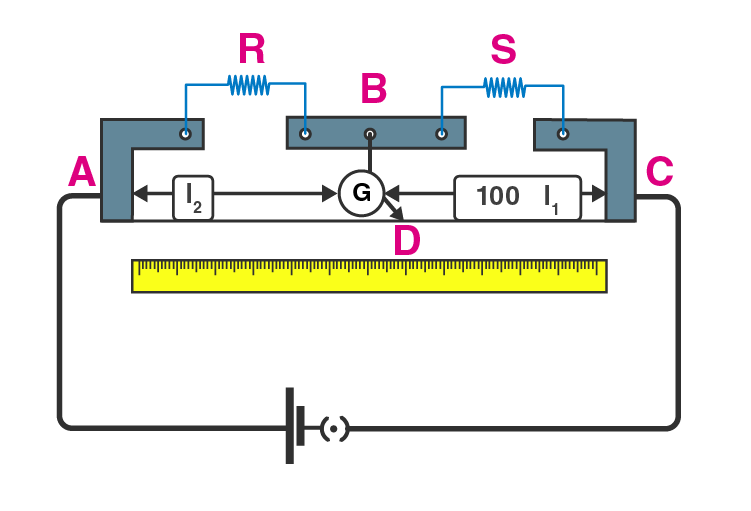a) the meter bridge can have no other neutral point for this set of resistances

b) when the jockey contacts a point on meter wire left of D, current flows to B from the wire

c) when the jockey contacts a point on a meter wire to the right of D, current flows from B to the wire through the galvanometer

d) when R is increased, the neutral point shifts to left

a) the meter bridge can have no other neutral point for this set of resistances

c) when the jockey contacts a point on a meter wire to the right of D, current flows from B to the wire through the galvanometer

3.12. Is the momentum conserved when charge crosses a junction in an electric circuit? Why or why not?

The momentum is not conserved when the charge crosses a junction in an electric circuit. This is because the drift velocity is proportional to the electric field.

3.13. The relaxation time τ is nearly independent of applied E field whereas it changes significantly with temperature T. First fact is responsible for Ohm’s law whereas the second fact leads to a variation of ρ with temperature. Elaborate why?

Relaxation time is the time interval between two successive collisions of the electrons.

It is defined as

τ = mean free path/rms velocity of electrons

Usually, the drift velocity of the electrons is small because there is a frequent collision between the electrons.

ρ = 1/σ = m/ne2τ

3.14. What are the advantages of the null-point method in a Wheatstone bridge? What additional measurements would be required to calculate R unknown by any other?

The advantage of a null-point in the Wheatstone bridge is that the resistance of the galvanometer is not affected by the balance point. The R unknown is calculated by using Kirchhoff’s rule.

3.15. What is the advantage of using thick metallic strips to join wires in a potentiometer?

The advantage of using thick metallic strips is that the resistance of these strips are negligible.

3.16. For wiring in the home, one uses Cu wires or Al wires. What considerations are involved in this?

The main considerations in the selection of the wires is the conductivity of the metal, cost of metal, and their availability.

3.17. Why are alloys used for making standard resistance coils?

Alloys are used in the making of the standard resistance coils because they have less temperature coefficient of resistance and the temperature sensitivity is also less.

3.18. Power P is to be delivered to a device via transmission cables having resistance Rc. If V is the voltage across R and I the current through it, find the power wasted and how can it be reduced.

Power consumed by the transmission lines is given as P = i2Rc

Where Rc is the resistance of connecting cables

Power is given as P = VI

The transmission of power takes place either during low voltage and high current or during high voltage and low current.

3.19. AB is a potentiometer wire. If the value of R is increased, in which direction will the balance point J shift?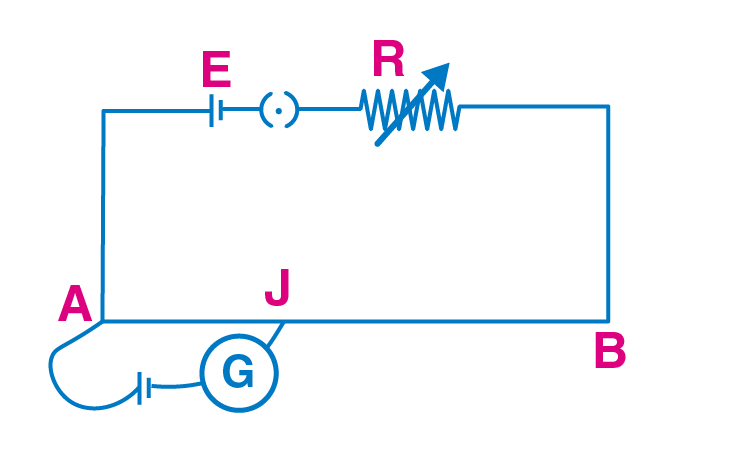When the value of R is increased, the potential difference across AB decreased and hence the potential gradient across AB decreases. This is given as E’ = kl

Jockey shifts towards B when the balance length l increases.

3.20. While doing an experiment with potentiometer it was found that the deflection is one-sided and i) the deflection decreased while moving from one end A of the wire to the end B; ii) the deflection increased while the jockey was moved towards end B.

i) Which terminal +ve or –ve of the cell E, is connected at X in case i) and how is E1 related to E?

ii) Which terminal of the cell E1 is connected at X in case ii)?

i) E is connected to the positive terminal of the cell E1 and it is connected to X. Also, E1 > E

ii) The negative terminal of the cell E1 is connected to X.

3.21. A cell of emf E and internal resistance r is connected across an external resistance R. Plot a graph showing the variation of PD across R versus R.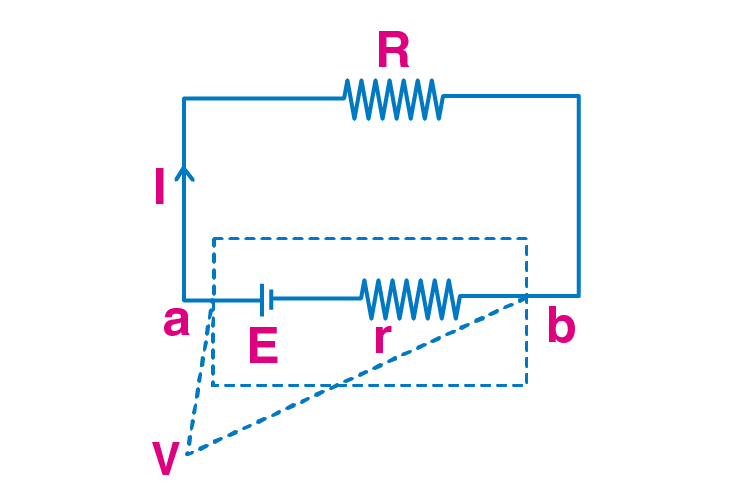E is the emf of the cell and r is the resistance which is connected across the external resistance R. The relationship between voltage and R is

V = ER/R+r

When R increases, V approaches closer to E and when E is infinite, V is zero.

Following is the graphical representation: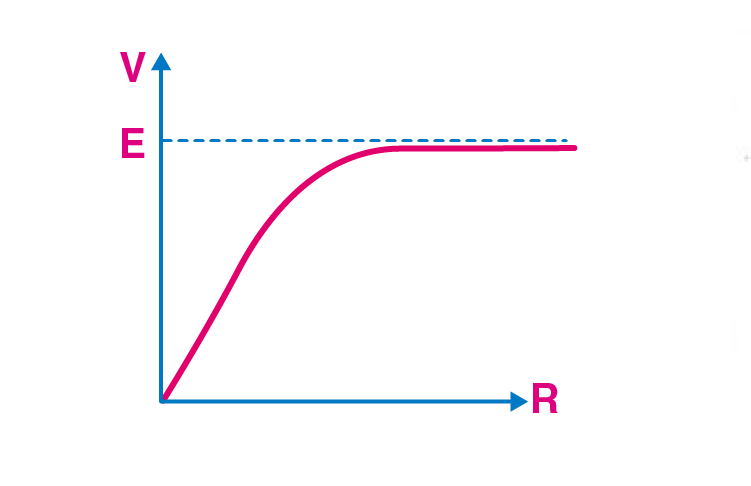3.22. First, a set of n equal resistors of R each are connected in series to a battery of emf E and internal resistance R. A current I is observed to flow. Then the n resistors are connected in parallel to the same battery. It is observed that the current is increased 10 times. What is ‘n’?

When the resistors are in series combination, the current is given as I = E/R+nR

When the resistors are in parallel combination, current is given as 10I = E/(R+R/n)

Solving the two equations, we get

n = 10

3.23. Let there be n resistors R1……..Rn with Rmax = max(R1……Rn) and Rmin = min(R1…….Rn). Show that when they are connected in parallel, the resultant resistance Rp < Rmin and when they are connected in series, the resultant resistance Rs > Rmax. Interpret the result physically.

Following is the parallel grouping of the resistance:

Rp = 1/R1 + 1/R2 + …. + 1/Rn

Rmin/Rp = Rmin/R1 + Rmin/R2 + …..+Rmin/Rn

The RHS is equal to 1

Therefore, Rp < Rmin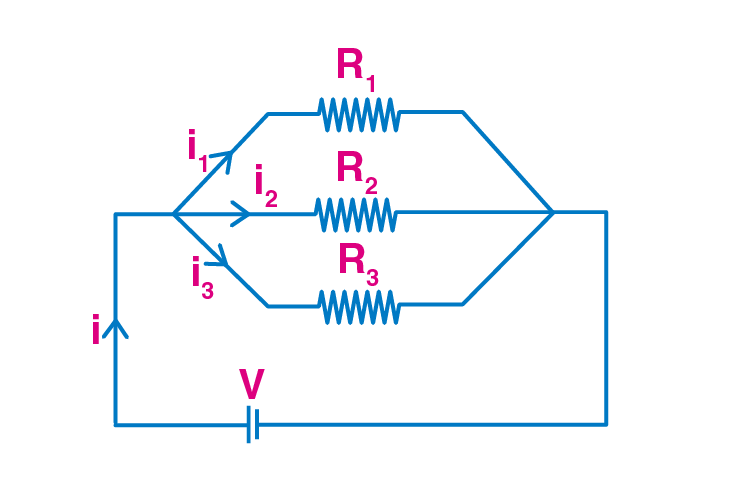Following is the series grouping of the resistance:

Rs = R1 + R2 + …… + Rn

Rs = R1 + ….. +Rmax+…..+Rn

Rs = Rmax(R1+……..Rn)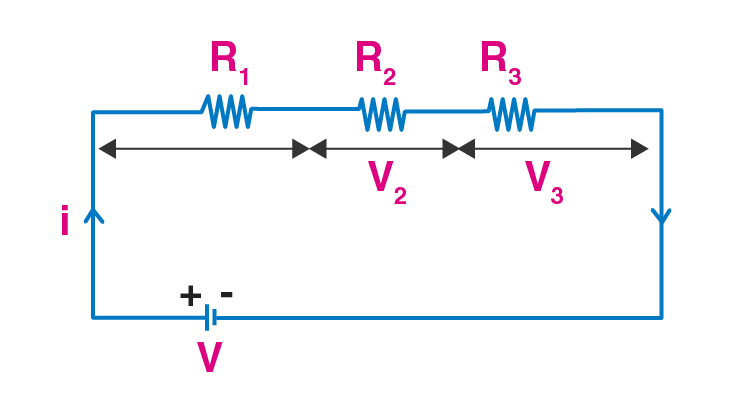Therefore, series combination has an equivalent resistance of resistors which is greater than the maximum resistance in parallel combination.

Physical interpretation is: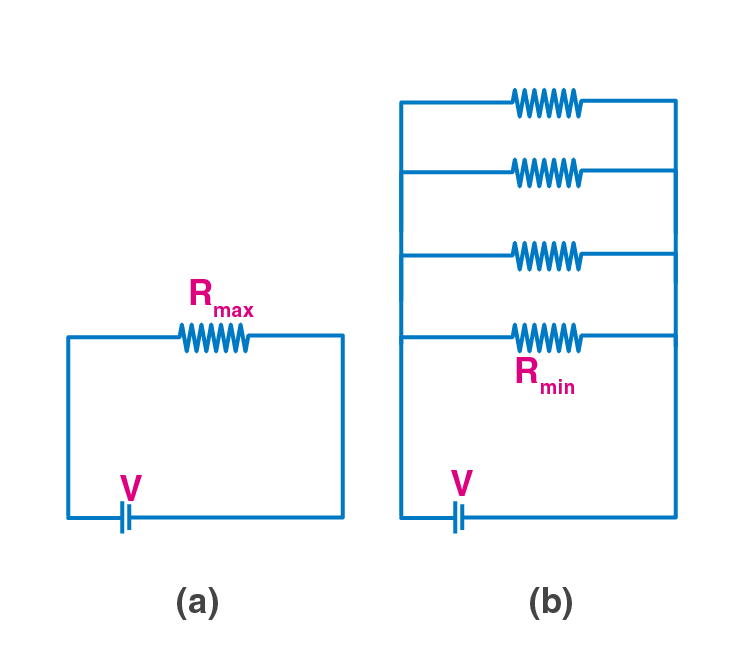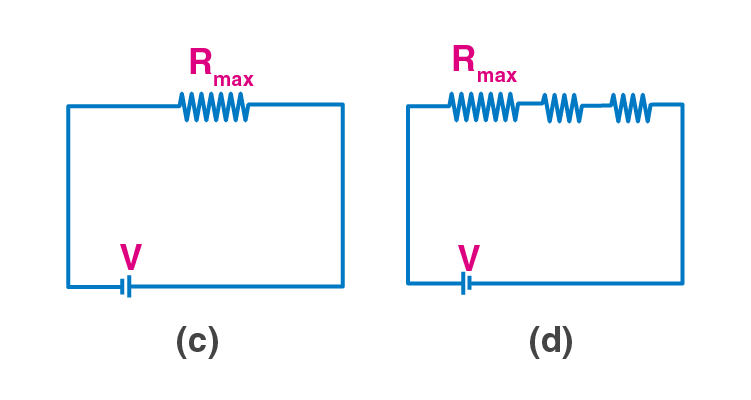3.24. The circuit in the figure shows two cells connected in opposition to each other. Cell E1 is of emf 6V and internal resistance 2Ω; the cell E2 is of emf 4V and internal resistance 8 Ω. Find the potential difference between the points A and B.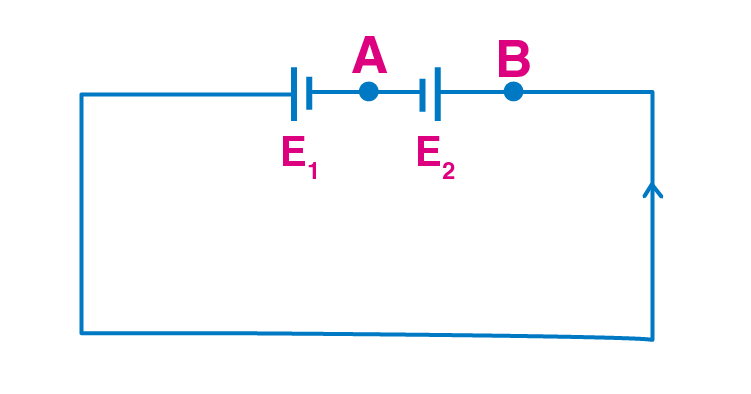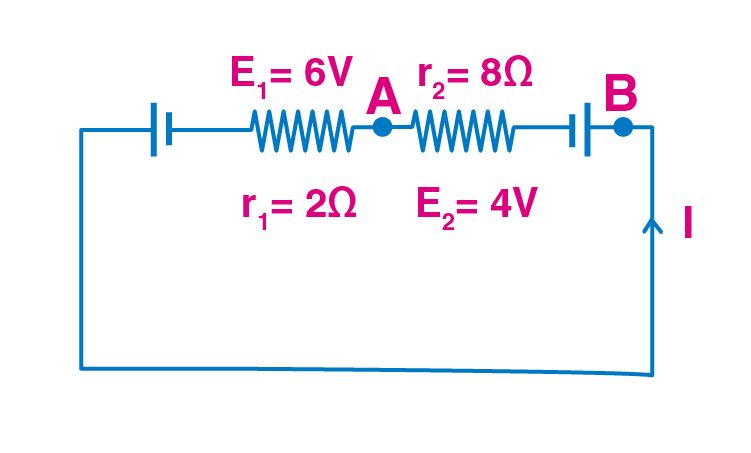Applying Ohm’s law, equivalent emf of the two cells = 6 – 4 = 2V

Equivalent resistance = 2 + 8 = 10 Ω

Electric current, I = 6-4/2+8 = 0.2A

When the loop is considered in the anti-clockwise direction, E1 > E2 which means VB > VA

Therefore, VB – VA = 3.6 V

3.25. Two cells of same emf E but internal resistance r1 and r2 are connected in series to an external resistor R. What should be the value of R so that the potential difference across the terminals of the first cell becomes zero.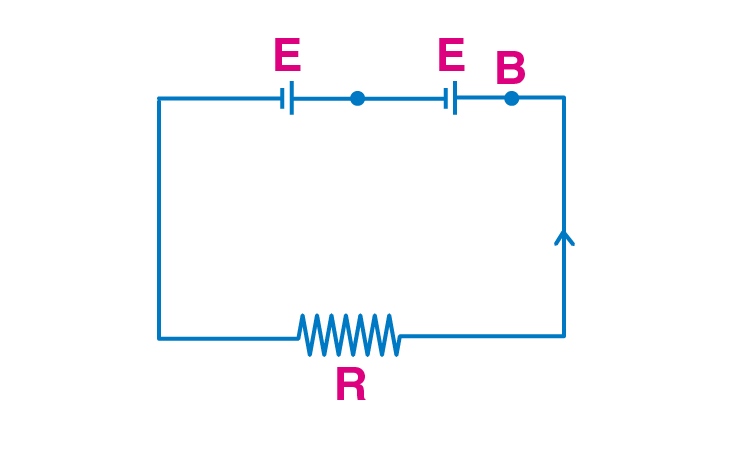Effective emf of two cells = E + E = 2E

Effective resistance = R + r1 + r2

Electric current is given as

I = 2E/R+r1+r2

Potential difference is given as

V1 – E – Ir1 = 0

Which f=gives R = r1 – r2

3.26. Two conductors are made of the same material and have the same length. Conductor A is a solid wire of diameter 1 mm. Conductor B is a hollow tube of outer diameter 2 mm and inner diameter 1 mm. Find the ratio of resistance RA to RB.

Resistance of wire R = ρ l/A

Where A is the cross-sectional area of the conductor

L is the length of the conductor

ρ is the specific resistance

RA = ρl/π(10-3 × 0.5)2

RB = ρl/ π[10-3)2 × (0.5 × 10-3)2]

Therefore, the ratio is RA/RB = 3:1

3.27. Suppose there is a circuit consisting of only resistances and batteries and we have to double all voltages and all resistances. Show that currents are unaltered.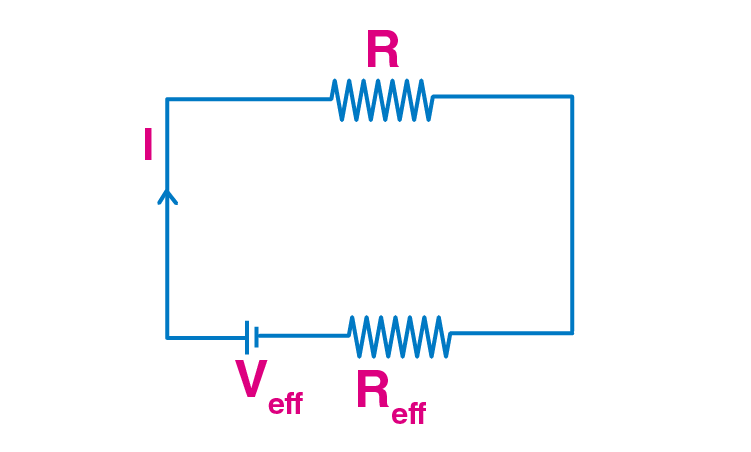Reff is the equivalent internal resistance of the battery

Veff is the equivalent voltage of the battery

Using Ohm’s law,

I = Veff/(Reff + R)

When the resistance and effective voltage are increased by n-times,

Veff = nVeff

Reff = n Reff

Rnew = nR

Then current is given as

I’ = bVeff/nReff + nR = Veff/Reff + R = I

3.28. Two cells of voltage 10V and 2V and internal resistances 10Ω and 5Ω respectively are connected in parallel with the positive end of the 10V battery connected to the negative pole of 2V battery. Find the effective voltage and effective resistance of the combination.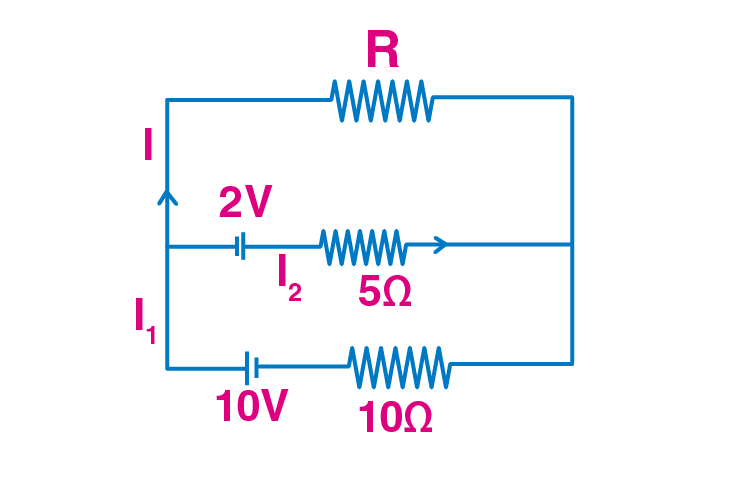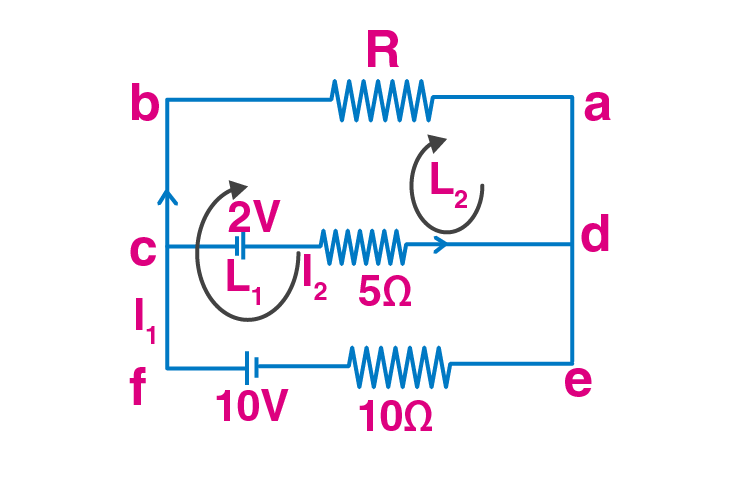Kirchhoff’s law is applied at c, I1 = I + I2

Kirchhoff’s law is applied at efbae, 10 – IR – 10I2 = 0

10 = IR + 10I1

Kirchhoff’s law is applied at cbadc, -2-IR+5I2 = 0

2 = 5I2- RI

I2 = I1 – I

Substituting the values we get

Equivalent internal resistance of the two cells as (10/3) Ohms

Following is the equivalent circuit when the combination of 5 and 10 Ohm are used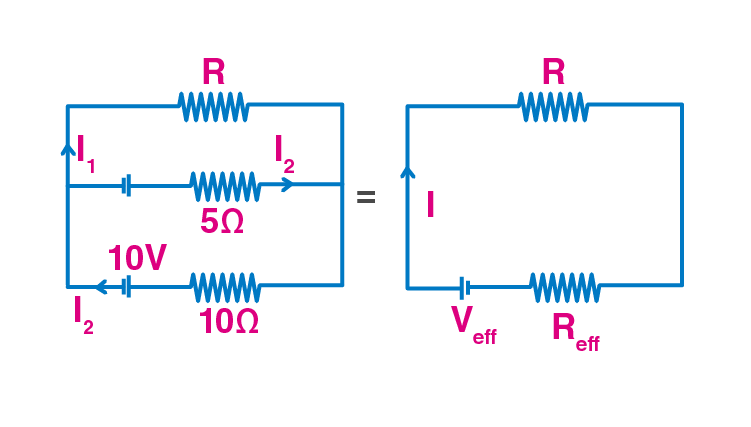3.29. A room has AC run for 5 hours a day at a voltage of 220V. The wiring of the room consists of Cu of 1 mm radius and a length of 10 m. Power consumption per day is 10 commercial units. What fraction of it goes in the joule heating in wires? What would happen if the wiring is made of aluminium of the same dimensions?

Power consumption in a day = 10 units

Power consumption per hour = 2 units

Power consumption = 2 units = 2 kW = 2000 J/s

Power consumption in resistors, P = VI

Which gives I = 9A

We know that resistance, R = ρ l/A

Substituting the values we get, resistance = 4 J/s

This is used in the calculation of power loss in Al wire = 6.4 J/s

Fractional loss in the second wire due to Joule heating is = 0.32%

3.30. In an experiment with a potentiometer, VB = 10V. R is adjusted to be 50 Ω. A student wanting to measure voltage E1 of a battery finds no null point possible. He then diminishes R to 10 Ω and is able to locate the null point on the last segment of the potentiometer. Find the resistance of the potentiometer wire and potential drop per unit length across the wire in the second case.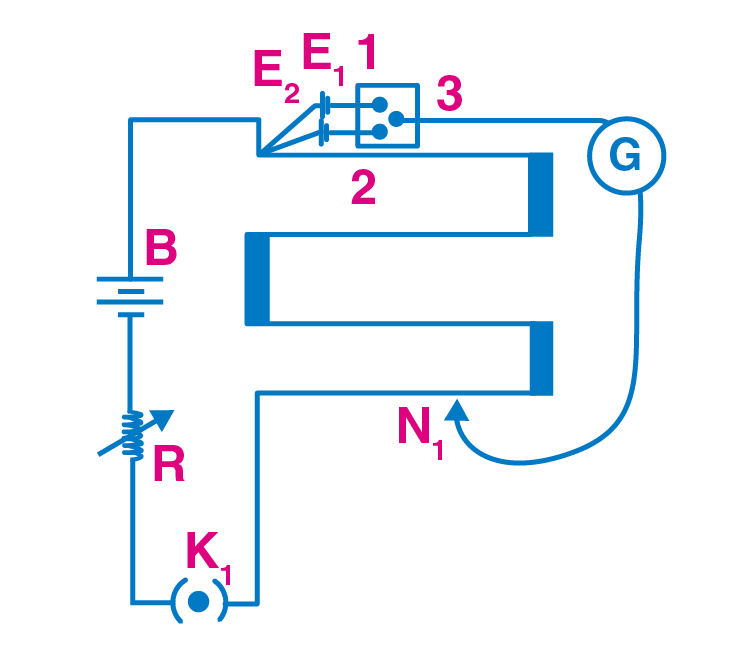Equivalent resistance of the potentiometer = 50 Ohm + R’

Equivalent voltage across the potentiometer = 10 V

Current through the main circuit I = 10/(50 Ohms +R’)

Potential difference across wire of potentiometer is:

When 50 ohms resistors is used

2R’ < 400

R’ < 200 Ohms

When 10 Ohm resistor is used

160 < R’ < 200

When the resistor is between 160 Ohms and 200 Ohms,

The potential gradient is given as

k > 2 V/m

When the potential drop across 300 cm wire is < 8 V

k < 2 2/3 V/m

3.31. a) Consider the circuit in the figure. How much energy is absorbed by electrons from the initial state of no current to the state of drift velocity?

b) Electrons give up energy at the rate of RI2 per second to the thermal energy. What time scale would one associate with energy in problem a) n = no of electron/volume = 1029/m3, length of circuit = 10 cm, cross-section = A = 1mm2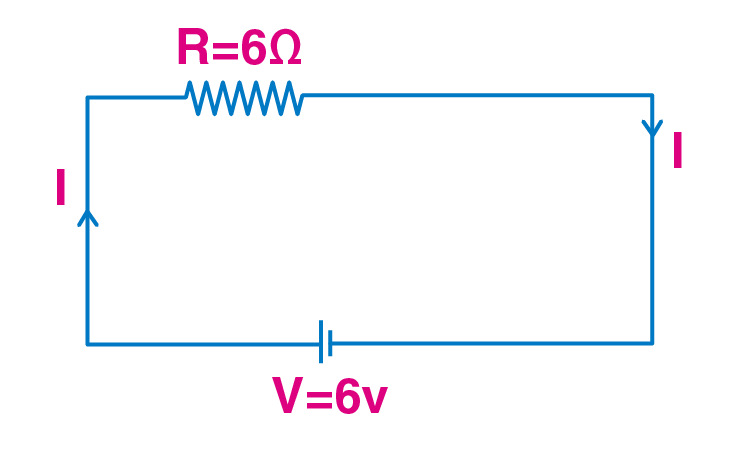a) Current is given as I = V/R from the Ohm’s law

Therefore, I = 1A

But, I = neAvd

vd = I/neA

When the values for the above parameters are substituted, vd = 1/1.6 × 10-4 m/s

The KE = (KE of one electron)(no.of electrons) = 2 × 10-17J

b) Power loss, P = I2R = 6 J/s

P = E/t

E = Pt

t = E/P = 10-17 s

Current electricity, which is also known as electric current, can simply be defined as the flow of electric charge that takes place. The charge that usually occurs takes place with the help of electrons moving through the wire. The charge present can be either ion in an electrolyte or through the ionised gas called plasma.

### Concepts Covered in NCERT Exemplar Solutions for Class 12 Physics Chapter 3 Current Electricity

 Section Number Topic 1 Electric Current – formula derivation, numerical 2 Electric Currents in Conductors 3 Ohm’s Law – definition, formula, problems 4 Drift of Electrons and the Origin of Resistivity 5 Mobility- definition, formula derivation and problems 6 Limitations of Ohm’s Law 7 Resistivity of Various Materials – resistivity chart, their usage in problems 8 Temperature Dependence of Resistivity – Derivation of resistivity at a temperature formula – Derivation of finite resistance formula and numerical 9 Electrical Energy, Power – circuit diagrams, derivations, numerical 10 Combination of Resistors – Series and parallel 11 Cells, Emf, Internal Resistance – circuit diagrams, derivations, numerical 12 Cells in Series and in Parallel 13 Kirchhoff’s Rules – statements, numerical 14 Wheatstone Bridge – application of Kirchhoff’s rules 15 Meter Bridge – introduction and application 16 Potentiometer

BYJU’S is India’s number one learning App with top-notch teachers on its board. BYJU’S provides video lessons that will help you retain the concepts and topics you have learnt for a long time, which is very essential in order to be successful in competitive examinations. BYJU’S – The Learning App guides you not only in understanding the concepts clearly but also in scoring high marks in the board examinations. For more information on NCERT Solutions and exemplar questions, download BYJU’S – The Learning App or visit BYJU’S website.